# Learn Go: The complete course

Hey, welcome to the course, and thanks for learning Go. I hope this course provides a great learning experience!

# Table of content

• Getting Started

• Chapter I

• Chapter II

• Chapter III

• Chapter IV

• Appendix

# What is Go?

Go (also known as Golang) is a programming language developed at Google in 2007 and open-sourced in 2009.

It focuses on simplicity, reliability, and efficiency. It was designed to combine the efficacy, speed, and safety of a statically typed and compiled language with the ease of programming of a dynamic language to make programming more fun again.

In a way, they wanted to combine the best parts of Python and C++ so that they can build reliable systems that can take advantage of multi-core processors.

# Why learn Go?

Before we start this course, let us talk about why we should learn Go

### 1. Easy to learnGo quite easy to learn and has a supportive and active community.

And being a multipurpose language you can use it for things like backend development, cloud computing, and more recently data science.

### 2. Fast and ReliableWhich makes it highly suitable for distributed systems. Projects such as Kubernetes and Docker are written in Go.

### 3. Simple yet powerfulGo has just 25 keywords which makes it easy to read, write and maintain. The language itself is concise.

But don't be fooled by the simplicity, Go has several powerful features that we will later learn in the course.

### 4. Career opportunitiesGo is growing fast and is being adopted by companies of any size. And with that new high-paying job opportunities.

I hope this made you excited about Go. Let's start this course.

In this tutorial, we will install Go and setup our code editor

# Installation and Setup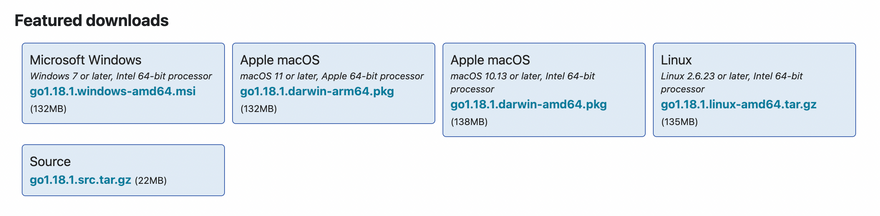## Installation

These instructions are from the official website

### MacOS

The package installs the Go distribution to `/usr/local/go`. The package should put the `/usr/local/go/bin` directory in your `PATH` environment variable.
You may need to restart any open Terminal sessions for the change to take effect.

2. Verify that you've installed Go by opening a command prompt and typing the following command:

``````\$ go version
``````
1. Confirm that the command prints the installed version of Go.

### Linux

1. Remove any previous Go installation by deleting the `/usr/local/go` folder (if it exists), then extract the archive you just downloaded into `/usr/local`, creating a fresh Go tree in `/usr/local/go`:
``````\$ rm -rf /usr/local/go && tar -C /usr/local -xzf go1.18.1.linux-amd64.tar.gz
``````

(You may need to run the command as root or through sudo)

Do not untar the archive into an existing `/usr/local/go` tree. This is known to produce broken Go installations.

1. Add `/usr/local/go/bin` to the PATH environment variable. You can do this by adding the following line to your `\$HOME/.profile` or `/etc/profile` (for a system-wide installation):
``````export PATH=\$PATH:/usr/local/go/bin
``````

Note: Changes made to a profile file may not apply until the next time you log into your computer. To apply the changes immediately, just run the shell commands directly or execute them from the profile using a command such as source `\$HOME/.profile`.

1. Verify that you've installed Go by opening a command prompt and typing the following command:
``````\$ go version
``````
1. Confirm that the command prints the installed version of Go.

### Windows

By default, the installer will install Go to Program Files or Program Files (x86).
You can change the location as needed. After installing, you will need to close and reopen any open command prompts so that changes to the environment made by the installer are reflected at the command prompt.

1. Verify that you've installed Go.
1. In Windows, click the Start menu.
2. In the menu's search box, type cmd, then press the Enter key.
3. In the Command Prompt window that appears, type the following command:
``````\$ go version
``````
1. Confirm that the command prints the installed version of Go.

## VS Code

In this course, I will be using VS Code and you can download it from here.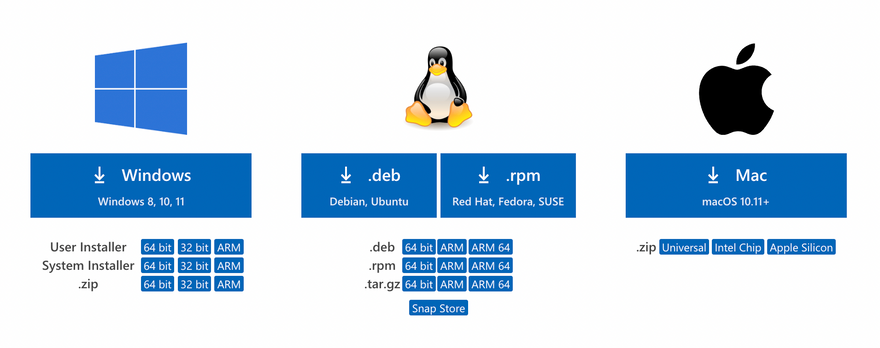Feel free to use any other code editor you prefer

### Extension

Make sure to also install the Go extension which makes is easier to work
with Go in VS Code.This is it for the installation and setup of Go, let's start the course and write our first hello world!

# Hello World

Let's write our first hello world program, we can start by initializing a module. For that, we can use the `go mod` command

``````\$ go mod init example
``````

But wait...what's a `module`? Don't worry we will discuss that soon! But for now, assume that the module is basically a collection of Go packages.

Moving ahead, Let's now create a `main.go` file and write a program that simply prints hello world.

``````package main

import "fmt"

func main() {
fmt.Println("Hello World!")
}
``````

If you're wondering, `fmt` is part of the Go standard library which is a set of core packages provided by the language

Now, let's quickly breakdown what we did here, or rather the structure of a go program

First, we defined a package such as `main`

``````package main
``````

Then, we have some imports

``````import "fmt"
``````

Last but not least is our `main` function which acts as an entry point for our application, just like in other languages like C, Java, or C#.

``````func main() {
...
}
``````

Remember, the goal here is to keep a mental note, and later in the course, we'll learn about `functions`, `imports`, and other things in detail!

Finally, to run our code, we can simply use `go run` command

``````\$ go run main.go
Hello World!
``````

Congratulations, you just wrote your first Go program!

With that let's move on to the next topic

# Variables and Data types

In this tutorial, we will learn about variables. We will also learn about the different data types that Go provides us.

## Variables

This is also known as declaration without initialization

``````var foo string
``````

Declaration with initialization

``````var foo string = "Go is awesome"
``````

Multiple declarations

``````var foo, bar string = "Hello", "World"
// OR
var (
foo string = "Hello"
bar string  = "World"
)
``````

Type is omitted but will be inferred

``````var foo = "What's my type?"
``````

Shorthand, here we omit `var` keyword and type is always implicit. This is how we will see variables being declared most of the time. We also use the `:=` for declaration plus assignment

``````foo := "Shorthand!"
``````

Note: Shorthand only works inside `function` bodies

## Constants

We can also declare constants with the `const` keyword. Which as the name suggests are fixed values that cannot be reassigned

``````const constant = "This is a constant"
``````

## Data Types

Perfect! Now let's look at some basic data types available in Go. Starting with string.

### String

In Go, a string is a sequence of bytes.

They are declared either using double quotes or backticks which can span multiple lines

``````var name string = "My name is Go"

var bio string = `I am statically typed.
``````

### Bool

Next is `bool` which is used to store boolean values. It can have two possible values - `true` or `false`.

``````var value bool = false
var isItTrue bool = true
``````

Operators

We can use the following operators on boolean types

Logical && !
Equality == !=

### Numeric types

Now let's talk about numeric types, starting with

Signed and Unsigned integers

Go has several built-in integer types of varying sizes for storing signed and unsigned integers

The size of generic `int` and `uint` type is platform dependent. Which means it is 32 bits wide on a 32-bit system and 64-bits wide on a 64-bit system.

``````var i int = 404                     // Platform dependent
var i8 int8 = 127                   // -128 to 127
var i16 int16 = 32767               // -2^15 to 2^15 - 1
var i32 int32 = -2147483647         // -2^31 to 2^31 - 1
var i64 int64 = 9223372036854775807 // -2^63 to 2^63 - 1
``````

Similar to signed integers, we have unsigned integers.

``````var ui uint = 404                     // Platform dependent
var ui8 uint8 = 255                   // 0 to 255
var ui16 uint16 = 65535               // 0 to 2^16
var ui32 uint32 = 2147483647          // 0 to 2^32
var ui64 uint64 = 9223372036854775807 // 0 to 2^64
var uiptr uintptr                     // Integer representation of a memory address
``````

If you noticed, there's also an unsigned integer pointer `uintptr` type, which is an integer representation of a memory address. It is not recommended to use this, so we don't have to worry about it.

So which one should we use?

It is recommended that whenever we need an integer value we should just use `int` unless we have a specific reason to use a sized or unsigned integer type.

Integer alias types

Next, let's discuss integer alias types.

Byte and Rune

Golang has two additional integer types called `byte` and `rune` that are aliases for `uint8` and `int32` data types respectively.

``````type byte = uint8
type rune = int32
``````

A `rune` represents a unicode code point.

``````var b byte = 'a'
var r rune = '🍕'
``````

Floating point

Next, we have floating point types which are used to store numbers with a decimal component.

Go has two floating point types `float32` and `float64`. Both types follow IEEE-754 standard.

The default type for floating point values is float64

``````var f32 float32 = 1.7812 // IEEE-754 32-bit
var f64 float64 = 3.1415 // IEEE-754 64-bit
``````

Operators

Go provides several operators for performing operations on numeric types.Complex

There are 2 complex types in Go. complex128 where both real and imaginary parts are float64 and complex64 where real and imaginary are float32.

We can define complex numbers either using the built-in complex function or as literals.

``````var c1 complex128 = complex(10, 1)
var c2 complex64 = 12 + 4i
``````

### Zero Values

Now let's discuss zero values. So in Go any variable declared without an explicit initial value are given their zero value. For example, let's declare some variables and see

``````var i int
var f float64
var b bool
var s string

fmt.Printf("%v %v %v %q\n", i, f, b, s)
``````
``````\$ go run main.go
0 0 false ""
``````

So, as we can see `int` and `float` are assigned as 0, `bool` as false, and `string` as an empty string. This is quite different from how other languages do it. For example, most languages initialize unassigned variables as null or undefined

This is great, but what are those percent symbols in our `Printf` function? As you've already guessed, they are used for formatting and we will learn about them in the next tutorial.

### Type Conversion

Moving on, now that we have seen how data types work, let's see how to do type conversion.

``````i := 42
f := float64(i)
u := uint(f)

fmt.Printf("%T %T", f, u)
``````
``````\$ go run main.go
float64 uint
``````

And as we can see, it prints the type as `float64` and `uint`.

Note that this is different from parsing

### Alias types

Alias types were introduced in Go 1.9

They allow developers to provide an alternate name for an existing type and use it interchangeably with the underlying type.

``````package main

import "fmt"

type MyAlias = string

func main() {
var str MyAlias = "I am an alias"

fmt.Printf("%T - %s", str, str) // Output: string - I am an alias
}
``````

### Defined types

Lastly, we have defined types that unlike alias types do not use an equals sign.

``````package main

import "fmt"

type MyDefined string

func main() {
var str MyDefined = "I am defined"

fmt.Printf("%T - %s", str, str) // Output: main.MyDefined - I am defined
}
``````

But wait...What's the difference?

So, defined types do more than just give a name to a type.

It first defines a new named type with an underlying type. However, this defined type is different from any other type, including its underline type.

Hence, it cannot be used interchangeably with the underlying type like alias types.

It's a bit confusing at first, hopefully, this example will make things clear.

``````package main

import "fmt"

type MyAlias = string

type MyDefined string

func main() {
var alias MyAlias
var def MyDefined

// ✅ Works
var copy1 string = alias

// ❌ Cannot use str (variable of type MyDefined) as string value in variable
var copy2 string = def

fmt.Println(copy1, copy2)
}
``````

As we can see, we cannot use the defined type interchangeably with the underlying type, unlike alias types.

Well, this is pretty much it for variables and data types in Go. I'll see you in the next one.

# String Formatting

In this tutorial, we will learn about string formatting or sometimes also known as templating.

`fmt` package contains lots of functions. So to save time, we will discuss the most frequently used functions. Let's start with `fmt.Print` inside our main function.

``````...

fmt.Print("What", "is", "your", "name?")
fmt.Print("My", "name", "is", "golang")
...
``````
``````\$ go run main.go
Whatisyourname?Mynameisgolang
``````

As we can see, `Print` does not format anything, it simply takes a string and prints it

Next, we have `Println` which is the same as `Print` but it adds a new line at the end and also inserts space between the arguments

``````...

fmt.Println("What", "is", "your", "name?")
fmt.Println("My", "name", "is", "golang")
...
``````
``````\$ go run main.go
My name is golang
``````

That's much better!

Next, we have `Printf` also known as “Print Formatter”, which allows us to format numbers, strings, booleans, and much more.

Let's look at an example

``````...
name := "golang"

fmt.Printf("My name is %s", name)
...
``````
``````\$ go run main.go
My name is golang
``````

As we can see that `%s` was substituted with our `name` variable.

But the question is what is `%s` and what does it mean?

So these are called annotation verbs and they tell the function how to format the arguments. We can control things like width, types, and precision with these and there are lots of them. Here's a cheatsheet

Now let's quickly look at some more examples. Here we will try to calculate a percentage and print it to the console

``````...
percent := (3/5) * 100
fmt.Printf("%f", percent)
...
``````
``````\$ go run main.go
58.181818
``````

Let's say we want just `58.18` which is 2 points precision, we can do that as well by using `.2f`

Also, to add an actual percent sign we will need to escape it.

``````...
percent := (3/5) * 100
fmt.Printf("%.2f %%", percent)
...
``````
``````\$ go run main.go
58.18 %
``````

This brings us to `Sprint`, `Sprintln`, and `Sprintf`. These are basically the same as the print functions, the only difference being they return the string instead of printing it.

Let's take an example

``````...
s := fmt.Sprintf("hex:%x bin:%b", 10 ,10)
fmt.Println(s)
...
``````
``````\$ go run main.go
hex:a bin:1010
``````

So, as we can see `Sprintf` formats our integer as hex or binary and returns it as a string.

Lastly, we have multiline string literals, which can be used like this

``````...
msg := `
Hello from
multiline
`

fmt.Println(msg)
...
``````

Great! But this is just the tip of the iceberg...so make sure to checkout the go doc for `fmt` package.

For those who are coming from C/C++ background, this should feel natural but if you're coming from let's say Python or JavaScript this might be a little strange at first. But it is very powerful and you'll see this functionality used quite extensively.

# Flow Control

Let's talk about flow control, starting with if/else.

## if/else

This works pretty much the same as you expect but the expression doesn't need to be surrounded by parentheses `()`

``````func main() {
x := 10

if x > 5 {
fmt.Println("x is gt 5")
} else if x > 10 {
fmt.Println("x is gt 10")
} else {
fmt.Println("else case")
}
}
``````
``````\$ go run main.go
x is gt 5
``````

### Compact if

We can also compact our if statements

``````func main() {
if x := 10; x > 5 {
fmt.Println("x is gt 5")
}
}
``````

Note: This pattern is quite common

## switch

Next, we have `switch` statement, which is often a shorter way to write conditional logic.

In Go, the switch case only runs the first case whose value is equal to the condition expression and not all the cases that follow. Hence, unlike other languages, `break` statement is automatically added at the end of each case.

This means that it evaluates cases from top to bottom, stopping when a case succeeds. Let's take an example

``````func main() {
day := "monday"

switch day {
case "monday":
fmt.Println("time to work!")
case "friday":
fmt.Println("let's party")
default:
fmt.Println("browse memes")
}
}
``````
``````\$ go run main.go
time to work!
``````

Switch also supports shorthand declaration like this

``````    switch day := "monday"; day {
case "monday":
fmt.Println("time to work!")
case "friday":
fmt.Println("let's party")
default:
fmt.Println("browse memes")
}
``````

We can also use the `fallthrough` keyword to transfer control to the next case even though the current case might have matched.

``````    switch day := "monday"; day {
case "monday":
fmt.Println("time to work!")
fallthrough
case "friday":
fmt.Println("let's party")
default:
fmt.Println("browse memes")
}
``````

And if we run this, we'll see that after the first case matches the switch statement continues to the next case because of the `fallthrough` keyword

``````\$ go run main.go
time to work!
let's party
``````

We can also use it without any condition, which is the same as `switch true`

``````x := 10

switch {
case x > 5:
fmt.Println("x is greater")
default:
fmt.Println("x is not greater")
}
``````

## Loops

Now, let's turn our attention toward loops.

So in Go, we only have one type of loop which is the `for` loop.

But it's incredibly versatile. Same as if statement, for loop, doesn't need any parenthesis `()` unlike other languages.

``````func main() {
for i := 0; i < 10; i++ {
fmt.Println(i)
}
}
``````

The basic `for` loop has three components separated by semicolons:

• init statement: which is executed before the first iteration
• condition expression: which is evaluated before every iteration
• post statement: which is executed at the end of every iteration

Break and continue

As expected, Go also supports both `break` and `continue` statements for loop control. Let's try a quick example

``````func main() {
for i := 0; i < 10; i++ {
if i < 2 {
continue
}

fmt.Println(i)

if i > 5 {
break
}
}

fmt.Println("We broke out!")
}
``````

So, the `continue` statement is used when we want to skip the remaining portion of the loop, and `break` statement is used when we want to break out of the loop.

Also, Init and post statements are optional, hence we can make our `for` loop behaves like a while loop as well.

``````func main() {
i := 0

for ;i < 10; {
i += 1
}
}
``````

Note: we can also remove the additional semi-colons to make it a little cleaner

### Forever loop

Lastly, If we omit the loop condition it loops forever, so an infinite loop can be compactly expressed. This is also known as the forever loop

``````func main() {
for {
// do stuff here
}
}
``````

Well, this is pretty much it on flow control, I'll see you in the next one!

# Functions

In this tutorial, we will discuss how we work with functions in Go. So, let's start with a simple function declaration

### Simple declaration

``````func myFunction() {}
``````

And we can call or execute it as follows

``````...
myFunction()
...
``````

Let's pass some parameters to it

``````func main() {
myFunction("Hello")
}

func myFunction(p1 string) {
fmt.Printtln(p1)
}
``````
``````\$ go run main.go
``````

As we can see it prints our message

We can also do a short hand declaration if the consecutive parameters have the same type. For example,

``````func myNextFunction(p1, p2 string) {}
``````

### Returning the value

Now let's also return a value

``````func main() {
s := myFunction("Hello")
fmt.Println(s)
}

func myFunction(p1 string) string {
msg := fmt.Sprintf("%s function", p1)
return msg
}
``````

### Multiple returns

Why return 1 value at a time, when we can do more? Go also supports multiple returns!

``````func main() {
s, i := myFunction("Hello")
fmt.Println(s, i)
}

func myFunction(p1 string) (string, int) {
msg := fmt.Sprintf("%s function", p1)
return msg, 10
}
``````

### Named returns

Another cool feature is named returns, where return values can be named and treated as their own variables

``````func myFunction(p1 string) (s string, i int) {
s = fmt.Sprintf("%s function", p1)
i = 10

return
}
``````

Notice how we added a `return` statement without any arguments, this is also known as naked return

I will say that, although this feature is interesting, please use it with care as this might reduce readability for larger functions

### Functions as values

Next, let's talk about functions as values, in Go functions are first class and we can use them as values. So let's clean up our function and try it out!

``````func myFunction() {
fn := func() {
fmt.Println("inside fn")
}

fn()
}
``````

We can also simplify this by making `fn` an anonymous function

``````func myFunction() {
func() {
fmt.Println("inside fn")
}()
}
``````

Notice how we execute it using the parenth*es*is at the end

### Closures

Why stop there? let's also return a function and hence create something called a closure. A simple definition can be that a closure is a function value that references variables from outside its body.

Closures are lexically scoped, which means functions can access the values in scope when defining the function.

``````func myFunction() func(int) int {
sum := 0

return func(v int) int {
sum += v

return sum
}
}
``````
``````...

...
``````

As we can see, we get a result of 15 as `sum` variable is bound to the function. This is a very powerful concept and definitely, a must know

Now let's look at variadic functions, which are functions that can take zero or multiple arguments using the `...` ellipses operator.

An example here would be a function that can add a bunch of values

``````func main() {
sum := add(1, 2, 3, 5)
fmt.Println(sum)
}

sum := 0

for _, v := range values {
sum += v
}

return sum
}
``````

Pretty cool huh? Also, don't worry about the `range` keyword, we will discuss it later in the course.

Fun fact: `fmt.Println` is a variadic function, that's how we were able to pass multiple values to it.

### defer

Lastly, let's discuss the `defer` keyword, which lets us postpones the execution of a function until the surrounding function returns.

``````func main() {
defer fmt.Println("I am finished")
fmt.Println("Doing some work...")
}
``````

Can we use multiple defer functions? Absolutely, this brings us to what is known as defer stack, let's take an example

``````func main() {
defer fmt.Println("I am finished")
defer fmt.Prinlnt("Are you?")

fmt.Println("Doing some work...")
}
``````
``````\$ go run main.go
Doing some work...
Are you?
I am finished
``````

As we can see, defer statements are stacked and executed in a last in first out manner.

So, Defer is incredibly useful and is commonly used for doing cleanup or error handling.

Functions can also be used with generics but we will discuss them later in the course.

So this is it for functions in go, see you in the next tutorial.

# Modules

In this tutorial, we will learn about modules.

### So what are modules?

Simply defined, A module is a collection of Go packages stored in a file tree with a `go.mod` file at its root, provided the directory is outside `\$GOPATH/src`

Go modules were introduced in Go 1.11, which brings native support for versions and modules. Earlier we needed the `GO111MODULE=on` flag to turn on the modules functionality when it was experimental but now after Go 1.13 modules mode is the default for all development

But wait what is `GOPATH`?

Well, `GOPATH` is a variable that defines the root of your workspace and it contains the following folders:

• src: contains Go source code organized in a hierarchy
• pkg: contains compiled package code
• bin: contains compiled binaries and executables.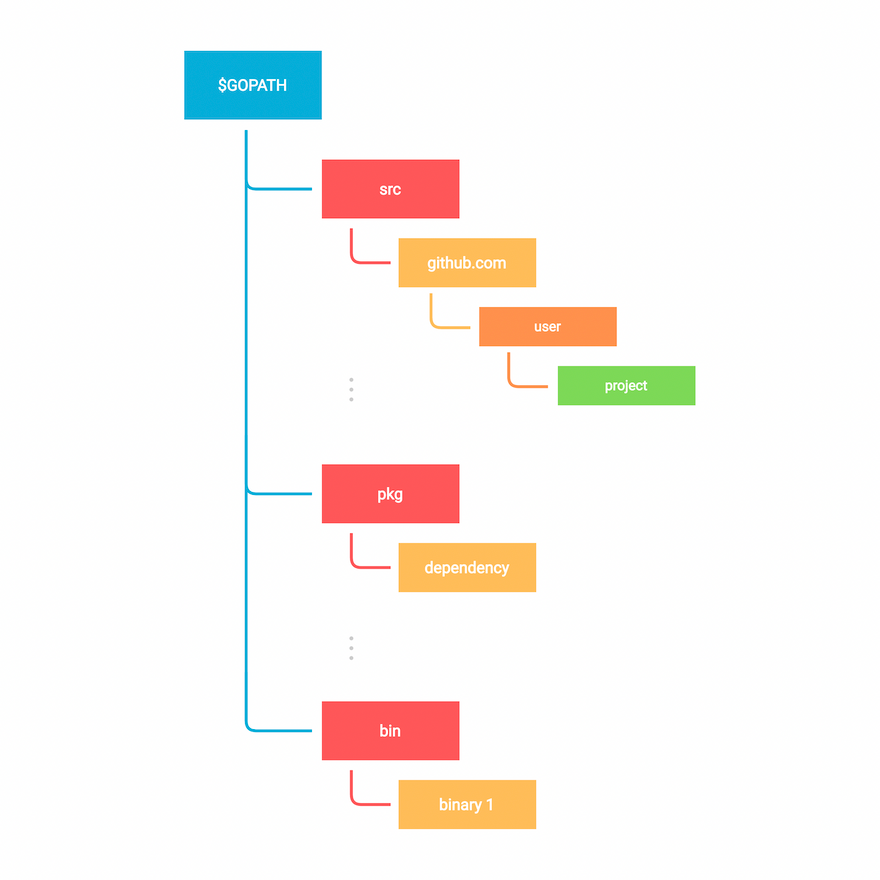Like earlier, let's create a new module using `go mod init` command which creates a new module and initializes the `go.mod` file that describes it

``````\$ go mod init example
``````

The important thing to note here is that a Go module can correspond to a Github repository as well if you plan to publish this module. For example,

``````\$ go mod init example
``````

Now, let's explore `go.mod` which is the file that defines the module's module path and also the import path used for the root directory, and its dependency requirements

``````module <name>

go <version>

require (
...
)
``````

And if we want to add a new dependency, we will use `go install` command

``````\$ go install github.com/rs/zerolog
``````

As we can see a `go.sum` file was also created. This file contains the expected hashes of the content of the new modules.

We can list all the dependencies using `go list` command as follows

``````\$ go list -m all
``````

If the dependency is not used, we can simply remove it using `go mod tidy` command.

``````\$ go mod tidy
``````

Finishing up our discussion on modules, let's also discuss vendoring.

Vendoring is the act of making your own copy of the 3rd party packages your project is using. Those copies are traditionally placed inside each project and then saved in the project repository.

This can be done through `go mod vendor` command

So let's reinstall the removed module using `go mod tidy`

``````package main

import "github.com/rs/zerolog/log"

func main() {
log.Info().Msg("Hello")
}
``````
``````\$ go mod tidy
go: finding module for package github.com/rs/zerolog/log
``````
``````\$ go mod vendor
``````
``````├── go.mod
├── go.sum
├── go.work
├── main.go
└── vendor
├── github.com
│   └── rs
│       └── zerolog
│           └── ...
└── modules.txt
``````

So this is pretty much it for modules, I'll see you in the next tutorial.

# Workspaces

In this tutorial, we will learn about multi-module workspaces that were introduced in Go 1.18

Workspaces allow us to work with multiple modules simultaneously without having to edit `go.mod` files for each module. Each module within a workspace is treated as a root module when resolving dependencies.

To understand this better, let's start by creating a `hello` module

``````\$ mkdir workspaces && cd workspaces
\$ mkdir hello && cd hello
\$ go mod init hello
``````

For demonstration purposes, I will add a simple `main.go` and install an example package.

``````package main

import (
"fmt"

"golang.org/x/example/stringutil"
)

func main() {
result := stringutil.Reverse("Hello Workspace")
fmt.Println(result)
}
``````
``````\$ go get golang.org/x/example
``````

And if we run this, we should see our output in reverse.

``````\$ go run main.go
ecapskroW olleH
``````

This is great, but what if we want to modify the `stringutil` module that our code depends on?

Until now we had to do it using the `replace` directive in the `go.mod` file, but now let's see how we can use workspaces here.

So let's create our workspace in the `workspace` directory

``````\$ go work init
``````

This will create a `[go.work](http://go.work)` file

``````\$ cat go.work
go 1.18
``````

We will also add our `hello` module to the workspace.

``````\$ go work use ./hello
``````

This should update the `[go.work](http://go.work)` file with a reference to our `hello` module

``````go 1.18

use ./hello
``````

Now let's download and modify the `stringutil` package and update the `Reverse` function implementation

``````\$ git clone https://go.googlesource.com/example
Cloning into 'example'...
remote: Total 204 (delta 39), reused 204 (delta 39)
Receiving objects: 100% (204/204), 467.53 KiB | 363.00 KiB/s, done.
Resolving deltas: 100% (39/39), done.
``````

`example/stringutil/reverse.go`

``````func Reverse(s string) string {
return fmt.Sprintf("I can do whatever!! %s", s)
}
``````

Finally, let's add `example` package to our workspace

``````\$ go work use ./example
\$ cat go.work
go 1.18

use (
./example
./hello
)
``````

Perfect, now if we run our `hello` module we will notice that the `Reverse` function has been modified.

``````\$ go run hello
I can do whatever!! Hello Workspace
``````

This is a very underrated feature from Go 1.18 but it is quite useful in certain circumstances.

So this is pretty much it for workspaces in Go, I'll see you in the next tutorial.

# Packages

In this tutorial, we will talk about packages.

### So what are packages?

A package is nothing but a directory containing one or more Go source files, or other Go packages.

This means every Go source file must belong to a package and package declaration is done at top of every source file as follows

``````package <package_name>
``````

So far we've done everything inside of `package main`. By convention, executable programs (by that I mean the ones with the `main` package) are called *Commands, o*thers are simply called Packages

The `main` package should also contain a `main()` function which is a special function that acts as the entry point of an executable program.

Let's take an example by creating our own package `custom` and adding some source files to it such as `code.go`

``````package custom
``````

Before we proceed any further, we should talk about imports and exports. Just like other languages, go also has a concept of imports and exports but it's very elegant.

Basically, any value (like a variable or function) can be exported and visible from other packages if they have been defined with an upper case identifier.

Let's try an example in our `custom` package

``````package custom

var value int = 10 // Will not be exported
var Value int = 20 // Will be exported
``````

As we can see lower case identifiers will not be exported and will be private to the package it's defined in. In our case the `custom` package.

That's great but how do we import or access it? Well, same as we've been doing so far unknowingly. Let's go to our `main.go` file and import our `custom` package.

Here we can refer to it using the `module` we had initialized in our `go.mod` file earlier

``````---go.mod---
module example

go 1.18

---main.go--
package main

import "example/custom"

func main() {
custom.Value
}
``````

Notice how the package name is the last name of the import path

We can import multiple packages as well like this.

``````package main

import (
"fmt"

"example/custom"
)

func main() {
fmt.Println(custom.Value)
}
``````

We can also alias our imports to avoid collisions like this

``````package main

import (
"fmt"

abcd "example/custom"
)

func main() {
fmt.Println(abcd.Value)
}
``````

External Dependencies

In Go, we are not only limited to working with local packages, we can also install external packages using `go install` command as we saw earlier.

So let's download a simple logging package `github.com/rs/zerolog/log`

``````\$ go install github.com/rs/zerolog
``````
``````package main

import (
"github.com/rs/zerolog/log"

abcd "example/custom"
)

func main() {
log.Print(abcd.Value)
}
``````

Also, make sure to checkout the go doc of packages you install, which is usually located in the project's readme file. go doc parses the source code and generates documentation in HTML format. Reference to It is usually located in readme files.

Lastly, I will add that, Go doesn't have a particular “folder structure” convention, always try to organize your packages in a simple and intuitive way.

So this is pretty much it for packages, see you in the next tutorial!

# Useful Commands

During our module discussion, we discussed some go commands related to go modules, let's now discuss some other important commands

Starting with `go fmt`, which formats the source code and it's enforced by that language so that we can focus on how our code should work rather than how our code should look.

``````\$ go fmt
``````

This might seem a little weird at first especially if you're coming from a javascript or python background like me but frankly, it's quite nice not to worry about linting rules.

Next, we have `go vet` which reports likely mistakes in our packages.

So if I go ahead and make a mistake in the syntax, and then run `go vet`

It should notify me of the errors

``````\$ go vet
``````

Next, we have `go env` which simply prints all the go environment information, we'll learn about some of these build-time variable in the next tutorial.

Lastly, we have, `go doc` which shows documentation for package or symbol, here's an example of the format package

``````\$ go doc -src fmt Printf
``````

Let's use `go help` command to see what other commands are available.

``````\$ go help
``````

As we can see, we have

`go fix` finds Go programs that use old APIs and rewrites them to use newer ones

`go generate` is usually used for code generation

`go install` compiles and install packages and dependencies

`go clean` is used for cleaning files that are generated by compilers

Some other very important commands are `go build` and `go test` but we will learn about them in detail later in the course.

This is pretty much it on go commands, feel free to experiment with them! And I'll see you in the next tutorial.

# Build

Building static binaries is one of the best features of Go which enables us to ship our code efficiently

We can do this very easily using the `go build` command

``````package main

import "fmt"

func main() {
fmt.Println("I am a binary!")
}
``````
``````\$ go build
``````

This should produce a binary with the name of our module. For example, here we have `example`

We can also specify the output

``````\$ go build -o app
``````

Now to run this, we simply need to execute it

``````\$ ./app
I am a binary!
``````

Yes, it's as simple as that!

Now, let's talk about some important build time variables, starting with

• `GOOS` and `GOARCH`

these environment variables help use build go programs for different operating systems
and underlying processor architectures

We can list all the supported architecture using `go tool` command

``````\$ go tool dist list
android/amd64
ios/amd64
js/wasm
linux/amd64
windows/arm64
.
.
.
``````

Here's an example for building a window's executable from macOS!

``````\$ GOOS=windows GOARCH=amd64 go build -o app.exe
``````
• `CGO_ENABLED`

This variable allows us to configure CGO which is a way in Go to call C code.

This helps us to produce statically linked binary that works without any external dependencies.

This is quite helpful for let's say when we want to run our go binaries in a docker container with minimum external dependencies.

Here's an example of how to use it

``````\$ CGO_ENABLED=0 go build -o app
``````

# Pointers

In this tutorial, we will discuss pointers. So what are Pointers?

Simply defined, a Pointer is a variable that is used to store the memory address of another variable.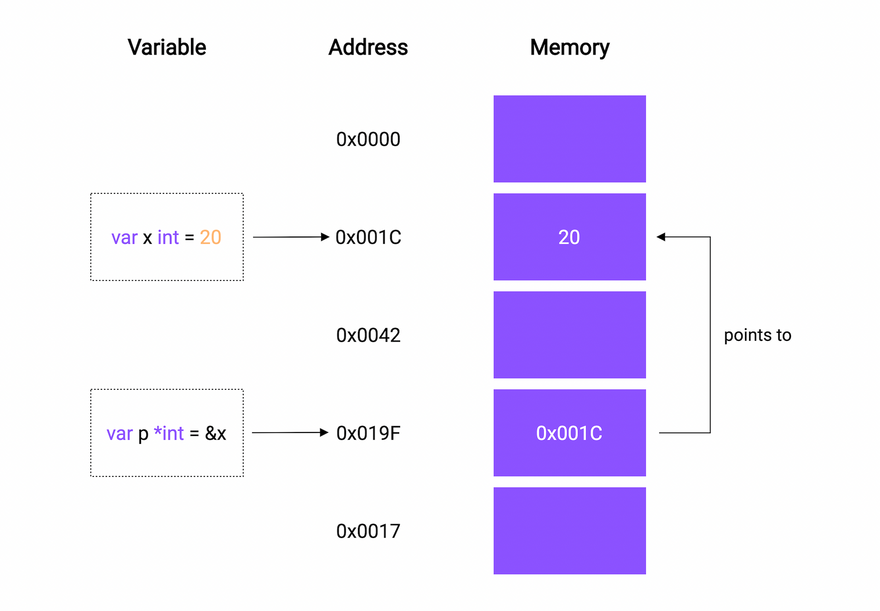It can be used like this

``````var x *T
``````

Where T is the type such as `int`, `string`, `float`, and so on

Let's try a simple example and see it in action

``````package main

import "fmt"

func main() {
var p *int

fmt.Println(p)
}
``````
``````\$ go run main.go
nil
``````

Hmm, this prints `nil`, but what is `nil`?

So nil is a predeclared identifier in Go that represents zero value for pointers, interfaces, channels, maps, and slices.

This is just like what we learned in the variables and datatypes section where we saw that uninitialized `int` has a zero value of 0, a `bool` has false, and so on.

Okay, now let's assign a value to the pointer

``````package main

import "fmt"

func main() {
a := 10

var p *int = &a

}
``````

We use the `&` ampersand operator to refer to a variable's memory address.

``````\$ go run main.go
0xc0000b8000
``````

This must be the value of the memory address of the variable `a`

## Dereferencing

We can also use the `*` asterisk operator to value stored in the variable that the pointer points to. This is called dereferencing

For example, we can access the value of the variable `a` through the pointer `p` using that `*` asterisk operator.

``````package main

import "fmt"

func main() {
a := 10

var p *int = &a

fmt.Println("value:", *p)
}
``````
``````\$ go run main.go
value: 10
``````

We can not only access it but change it as well through the pointer

``````package main

import "fmt"

func main() {
a := 10

var p *int = &a

fmt.Println("before", a)

*p = 20
fmt.Println("after:", a)
}
``````
``````\$ go run main.go
before 10
after: 20
``````

I think this is pretty neat!

### Pointers as function args

Pointers can also be used as arguments for a function when we need to pass some data by reference.

Here's an example

``````myFunction(&a)
...

func myFunction(ptr *int) {}
``````

## New function

There's also another way to initialize a pointer. We can use the `new` function which takes a type as an argument, allocates enough memory to accommodate a value of that type, and returns a pointer to it

Here's an example

``````package main

import "fmt"

func main() {
p := new(int)
*p = 100

fmt.Println("value", *p)
}
``````
``````\$ go run main.go
value 100
``````

### Pointer to a Pointer

Here's an interesting idea...can we create a pointer to a pointer. And the answer is yes!

Yes, we can.

``````package main

import "fmt"

func main() {
p := new(int)
*p = 100

p1 := &p

fmt.Println("P value", *p, " address", p)
fmt.Println("P1 value", *p1, " address", p)

fmt.Println("Dereferenced value", **p1)
}
``````
``````\$ go run main.go
Dereferenced value 100
``````

Notice how the value of `p1` matches the address of `p`

Also, it is important to know that pointers in Go do not support pointer arithmetic like in C or C++.

``````    p1 := p * 2 // Compiler Error: invalid operation
``````

However, we can compare two pointers of the same type for equality using `==` operator

``````p := &a
p1 := &a

fmt.Println(p == p1)
``````

## But Why?

This brings us to the million-dollar question, why do we need pointers?

Well, there's no definite answer for that, and pointers are just another useful feature that helps us mutate our data efficiently without copying a large amount of data.

And can be applied to tons of use cases.

Lastly, I will add that If you are coming from a language with no notion of pointers, don't panic and try to form a mental model of how pointers work.

Perfect! we learned about pointers and their use cases, now let's move on to the next topic.

# Structs

In this tutorial, we will learn about structs.

So, A `struct` is a user-defined type that contains a collection of named fields. Basically, It is used to group related data together to form a single unit.

If you're coming from an objected-oriented background, think of structs as lightweight classes which that support composition but not inheritance.

## Defining

We can define a `struct` like this

``````type Person struct {}
``````

We use the `type` keyword to introduce a new type, followed by the name and then the `struct` keyword to indicate that we're defining a struct

Now let's give it some fields

``````type Person struct {
FirstName string
LastName  string
Age       int
}
``````

And, If the fields have the same type, we can collapse them as well

``````type Person struct {
FirstName, LastName string
Age                 int
}
``````

## Declaring and initializing

Now that we have our struct, we can declare it the same as other datatypes

``````func main() {
var p1 Person

fmt.Println("Person 1:", p1)
}
``````
``````\$ go run main.go
Person 1: {  0}
``````

As we can see, all the struct fields are initialized with their zero values. So the `FirstName` and `LastName` are set to `“”` empty string and `Age` is set to 0.

We can simply initialize it as “struct literal” also

``````func main() {
var p1 Person

fmt.Println("Person 1:", p1)

var p2 = Person{FirstName: "Karan", LastName: "Pratap Singh", Age: 22}

fmt.Println("Person 2:", p2)
}
``````

For readability, we can separate by new line but this will also require a trialing comma

``````    var p2 = Person{
FirstName: "Karan",
LastName:  "Pratap Singh",
Age:       22,
}
``````
``````\$ go run main.go
Person 1: {  0}
Person 2: {Karan Pratap Singh 22}
``````

We can also initialize only a subset of fields

``````func main() {
var p1 Person

fmt.Println("Person 1:", p1)

var p2 = Person{
FirstName: "Karan",
LastName:  "Pratap Singh",
Age:       22,
}

fmt.Println("Person 2:", p2)

var p3 = Person{
FirstName: "Tony",
LastName:  "Stark",
}

fmt.Println("Person 3:", p3)
}
``````
``````\$ go run main.go
Person 1: {  0}
Person 2: {Karan Pratap Singh 22}
Person 3: {Tony Stark 0}
``````

As we can see, the age field of person 3 has defaulted to the zero value.

## Without field name

Go structs also supports initialization without field names

``````func main() {
var p1 Person

fmt.Println("Person 1:", p1)

var p2 = Person{
FirstName: "Karan",
LastName:  "Pratap Singh",
Age:       22,
}

fmt.Println("Person 2:", p2)

var p3 = Person{
FirstName: "Tony",
LastName:  "Stark",
}

fmt.Println("Person 3:", p3)

var p4 = Person{"Bruce", "Wayne"}

fmt.Println("Person 4:", p4)
}
``````

But here's the catch, we will need to provide all the values during the initialization or it will fail

``````\$ go run main.go
# command-line-arguments
./main.go:30:27: too few values in Person{...}
``````
``````    var p4 = Person{"Bruce", "Wayne", 40}

fmt.Println("Person 4:", p4)
``````

We can also declare an anonymous struct

``````func main() {
var p1 Person

fmt.Println("Person 1:", p1)

var p2 = Person{
FirstName: "Karan",
LastName:  "Pratap Singh",
Age:       22,
}

fmt.Println("Person 2:", p2)

var p3 = Person{
FirstName: "Tony",
LastName:  "Stark",
}

fmt.Println("Person 3:", p3)

var p4 = Person{"Bruce", "Wayne", 40}

fmt.Println("Person 4:", p4)

var a = struct {
Name string
}{"Golang"}

fmt.Println("Anonymous:", a)
}
``````

## Accessing fields

let's clean up our example a bit and see how we can access individual fields

``````func main() {
var p = Person{
FirstName: "Karan",
LastName:  "Pratap Singh",
Age:       22,
}

fmt.Println("FirstName", p.FirstName)
}
``````

We can also create pointer to structs as well

``````func main() {
var p = Person{
FirstName: "Karan",
LastName:  "Pratap Singh",
Age:       22,
}

ptr := &p

fmt.Println((*ptr).FirstName)
fmt.Println(ptr.FirstName)
}
``````

Both statements are equal as in Go we don't need to explicitly dereference the pointer

We can also use the built-in `new` function

``````func main() {
p := new(Person)

p.FirstName = "Karan"
p.LastName = "Pratap Singh"
p.Age = 22

fmt.Println("Person", p)
}
``````
``````\$ go run main.go
Person &{Karan Pratap Singh 22}
``````

As a side note, two structs are equal if all their corresponding fields are equal as well

``````func main() {
var p1 = Person{"a", "b", 20}
var p2 = Person{"a", "b", 20}

fmt.Println(p1 == p2)
}
``````
``````\$ go run main.go
true
``````

## Exported fields

Now let's learn what is exported and unexported fields in a struct. Same as the rules for variables and functions, if a struct field is declared with a lower case identifier, it will not be exported and only be visible to the package it is defined in.

``````type Person struct {
FirstName, LastName  string
Age                  int
zipCode              string
}
``````

So, the `zipCode` field won't be exported. Also, the same goes for the `Person` struct if we rename it as `person` it won't be exported as well.

``````type person struct {
FirstName, LastName  string
Age                  int
zipCode              string
}
``````

## Embedding and composition

As we discussed earlier, Go doesn't necessarily support inheritance, but we can do something similar with embedding

``````type Person struct {
FirstName, LastName  string
Age                  int
}

type SuperHero struct {
Person
Power   int
}
``````

So our new struct will have all the properties of the original struct. And this should behave same as our normal struct.

``````func main() {
s := SuperHero{}

s.FirstName = "Bruce"
s.LastName = "Wayne"
s.Age = 40
s.Alias = "batman"

fmt.Println(s)
}
``````
``````\$ go run main.go
{{Bruce Wayne 40} batman}
``````

However, this is usually not recommended and in most cases, composition is preferred. So rather than embedding, we will just define it as a normal field.

``````type Person struct {
FirstName, LastName  string
Age                  int
}

type SuperHero struct {
Person  Person
Alias   string
}
``````

Hence, we can re-write our example with composition as well

``````func main() {
p := Person{"Bruce", "Wayne", 40}
s := SuperHero{p, "batman"}

fmt.Println(s)
}
``````
``````\$ go run main.go
{{Bruce Wayne 40} batman}
``````

Again there is no right or wrong here, but nonetheless, embedding comes in handy sometimes.

## Struct tags

A struct tag is just a tag that allows us to attach metadata information to the field which can be used for custom behavior using the `reflect` package.

Let's learn how we can define struct tags.

``````type Animal struct {
Name    string `key:"value1"`
Age     int    `key:"value2"`
}
``````

You will often find tags in encoding packages, such as XML, JSON, YAML, ORMs, and Configuration management.

Here's a tags example for JSON encoder.

``````type Animal struct {
Name    string `json:"name"`
Age     int    `json:"age"`
}
``````

## Properties

Finally, let's discuss the properties of structs.

Structs are value types. When we assign one `struct` variable to another, a new copy of the `struct` is created and assigned.

Similarly, when we pass a `struct` to another function, the function gets its own copy of the `struct`.

``````package main

import "fmt"

type Point struct {
X, Y float64
}

func main() {
p1 := Point{1, 2}
p2 := p1 // Copy of p1 is assigned to p2

p2.X = 2

fmt.Println(p1) // Output: {1 2}
fmt.Println(p2) // Output: {2 2}
}
``````

Empty struct occupies zero bytes of storage

``````package main

import (
"fmt"
"unsafe"
)

func main() {
var s struct{}
fmt.Println(unsafe.Sizeof(s)) // Output: 0
}
``````

Well, this wraps up our discussion on `structs`. Next, we will learn how to extend our structs with methods.

# Methods

Technically, Go is not an object-oriented programming language. It doesn't have classes, objects, and inheritance.

However, Go has types. And, you can define methods on types.

A method is nothing but a function with a special receiver argument. Let's see how we can declare methods

``````func (variable T) Name(params) (returnTypes) {}
``````

The receiver argument has a name and a type. It appears between the `func` keyword and the method name

For example, let's define a `Car` struct

``````type Car struct {
Name string
Year int
}
``````

Now let us define a method like `IsLatest` which will tell us if a car was manufactured within the last 5 years

``````func (c Car) IsLatest() bool {
return c.Year >= 2017
}
``````

As you can see, we can access the instance of `Car` using the receiver variable `c`. I like to think of it as `this` keyword from the object-oriented world.

Now we should be able to call this method after we initialize our struct, just like we do with classes in other languages

``````func main() {
c := Car{"Tesla", 2021}

fmt.Println("IsLatest", c.IsLatest())
}
``````

With a value receiver, the method operates on a copy of the value passed to it. Therefore, any modifications done to the receiver inside the methods are not visible to the caller.

For example, let's make another method called `UpdateName` which will update the name of the `Car`

``````func (c Car) UpdateName(name string) {
c.Name = name
}
``````

Now let's run this

``````func main() {
c := Car{"Tesla", 2021}

c.UpdateName("Toyota")
fmt.Println("Car:", c)
}
``````
``````\$ go run main.go
Car: {Tesla 2021}
``````

seems like the name wasn't updated, so now let's switch our receiver to pointer type and try again

``````func (c *Car) UpdateName(name string) {
c.Name = name
}
``````
``````\$ go run main.go
Car: {Toyota 2021}
``````

As expected, methods with pointer receivers can modify the value to which the receiver points. Such modifications are visible to the caller of the method as well.

### Properties

Let's also see some properties of the methods!

• Go is smart enough to interpret our function call correctly and hence, pointer receiver method calls are just syntactic sugar provided by Go for convenience.
``````(&c).UpdateName(...)
``````
• We can omit the variable part of the receiver as well if we're not using it
``````func (Car) UpdateName(...) {}
``````
• Methods are not limited to structs but can also be used with non-struct types as well
``````package main

import "fmt"

type MyInt int

func (i MyInt) isGreater(value int) bool {
return i > MyInt(value)
}

func main() {
i := MyInt(10)

fmt.Println(i.isGreater(5))
}
``````

### Why methods instead of functions?

So the question is, why methods instead of functions?

As always, there's no particular answer for this and in no way one is better than the other. Instead, they should be used appropriately when the situation arrives.

One thing I can think of right now is that methods can help us avoid naming conflicts.

Since a method is tied to a particular type, we can have the same method names for multiple receivers.

But generally, It might just come down to preference? such as “method calls are much easier to read and understand than function calls” or the other way around.

So this wraps up our discussion on methods, see you in the next tutorial

# Arrays and Slices

In this tutorial, we will learn about arrays and slices in Go.

## Arrays

### So what's an array?

An array is a fixed-size collection of elements of the same type. The elements of the array are stored sequentially and can be accessed using their `index`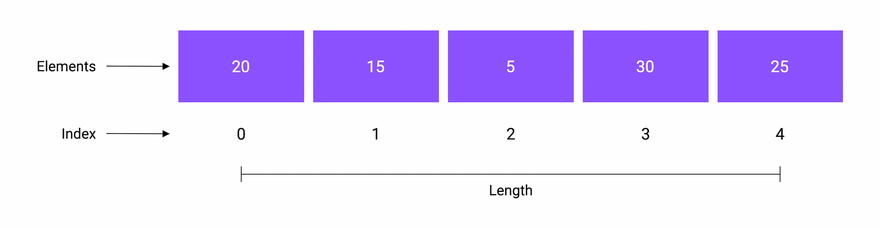### Declaration

We can declare an array as follows

``````var a [n]T
``````

Here `n` is the length and `T` can be any type like integer, string, or user-defined structs.

Now, let's declare an array of integers with length 4 and print it.

``````func main() {
var arr int

fmt.Println(arr)
}
``````
``````\$ go run main.go
[0 0 0 0]
``````

By default, all the array elements are initialized with the zero value of the corresponding array type.

### Initialization

We can also initialize an array using an array literal

``````var a [n]T = [n]T{V1, V2, ... Vn}
``````
``````func main() {
var arr = int{1, 2, 3, 4}

fmt.Println(arr)
}
``````
``````\$ go run main.go
[1 2 3 4]
``````

We can even do a shorthand declaration

``````...
arr := int{1, 2, 3, 4}
``````

### Access

And similar to other languages, we can access the elements using the `index` as they're stored sequentially

``````func main() {
arr := int{1, 2, 3, 4}

fmt.Println(arr)
}
``````
``````\$ go run main.go
1
``````

### Iteration

So there are multiple ways to iterate over arrays.

The first one is using the for loop with the `len` function which gives us the length of the array

``````func main() {
arr := int{1, 2, 3, 4}

for i := 0; i < len(arr); i++ {
fmt.Printf("Index: %d, Element: %d\n", i, arr[i])
}
}
``````
``````\$ go run main.go
Index: 0, Element: 1
Index: 1, Element: 2
Index: 2, Element: 3
Index: 3, Element: 4
``````

Another way is to use the `range` keyword with the for loop

``````func main() {
arr := int{1, 2, 3, 4}

for i, e := range arr {
fmt.Printf("Index: %d, Element: %d\n", i, e)
}
}
``````
``````\$ go run main.go
Index: 0, Element: 1
Index: 1, Element: 2
Index: 2, Element: 3
Index: 3, Element: 4
``````

As we can see our example works the same as before.

But the range keyword is quite versatile and can be used in multiple ways.

``````for i, e := range arr {} // Normal usage of range

for _, e := range arr {} // Omit index with _ and use element

for i := range arr {} // Use index only

for range arr {} // Simply loop over the array
``````

### Multi dimensional

All the arrays that we created so far are one dimensional. We can also create multi-dimensional arrays in Go.

Let's take an example

``````func main() {
arr := int{
{1, 2, 3, 4},
{5, 6, 7, 8},
}

for i, e := range arr {
fmt.Printf("Index: %d, Element: %d\n", i, e)
}
}
``````
``````\$ go run main.go
Index: 0, Element: [1 2 3 4]
Index: 1, Element: [5 6 7 8]
``````

we can also let the compiler infer the length of the array by using `...` ellipses instead of the length

``````func main() {
arr := [...]int{
{1, 2, 3, 4},
{5, 6, 7, 8},
}

for i, e := range arr {
fmt.Printf("Index: %d, Element: %d\n", i, e)
}
}
``````
``````\$ go run main.go
Index: 0, Element: [1 2 3 4]
Index: 1, Element: [5 6 7 8]
``````

### Properties

Now let's talk about some properties of arrays.

The array's length is part of its type. So, the array `a` and `b` are completely distinct types, and we cannot assign one to the other.

This also means that we cannot resize an array, because resizing an array would mean changing its type.

``````package main

func main() {
var a = int{1, 2, 3, 4}
var b int = a // Error, cannot use a (type int) as type int in assignment
}
``````

Arrays in Go are value types unlike other languages like C, C++, and Java where arrays are reference types.

This means that when we assign an array to a new variable or pass an array to a function, the entire array is copied.

So, if we make any changes to this copied array, the original array won't be affected and will remain unchanged.

``````package main

import "fmt"

func main() {
var a = string{"Mon", "Tue", "Wed", "Thu", "Fri", "Sat", "Sun"}
var b = a // Copy of a is assigned to b

b = "Monday"

fmt.Println(a) // Output: [Mon Tue Wed Thu Fri Sat Sun]
fmt.Println(b) // Output: [Monday Tue Wed Thu Fri Sat Sun]
}
``````

## Slices

I know what you're thinking, arrays are useful but a bit inflexible due to the limitation caused by their fixed size.

This brings us to Slice, so what is a slice?

A Slice is a segment of an array. Slices build on arrays and provide more power, flexibility, and convenience.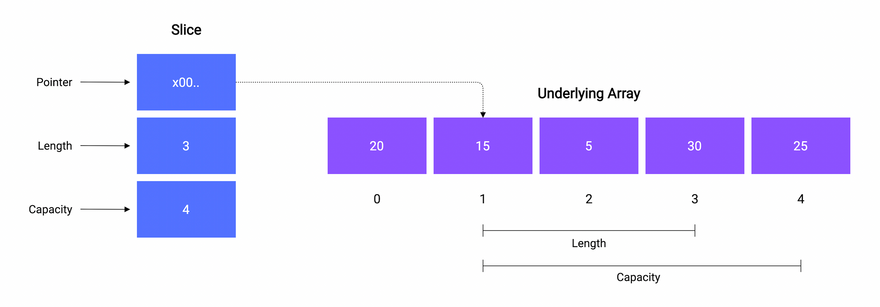A slice consists of three things:

• A pointer reference to an underlying array.
• The length of the segment of the array that the slice contains.
• And, the capacity, which is the maximum size up to which the segment can grow.

Just like `len` function, we can determine the capacity of a slice using the built-in `cap` function. Here's an example,

``````package main

import "fmt"

func main() {
a := int{20, 15, 5, 30, 25}

s := a[1:4]

// Output: Array: [20 15 5 30 25], Length: 5, Capacity: 5
fmt.Printf("Array: %v, Length: %d, Capacity: %d\n", a, len(a), cap(a))

// Output: Slice [15 5], Length: 3, Capacity: 4
fmt.Printf("Slice: %v, Length: %d, Capacity: %d", s, len(s), cap(s))
}
``````

Don't worry, we are going to discuss everything shown here in detail.

### Declaration

Let's see how we can declare a slice

``````var s []T
``````

As we can see, we don't need to specify any length

Let's declare a slice of integers and see how it works

``````func main() {
var s []string

fmt.Println(s)
fmt.Println(s == nil)
}
``````
``````\$ go run main.go
[]
true
``````

So, unlike arrays, the zero value of a slice is `nil`

### Initialization

There are multiple ways to initialize our slice. One way is to use the built-in `make` function

``````make([]T, len, cap) []T
``````
``````func main() {
var s = make([]string, 0, 0)

fmt.Println(s)
}
``````
``````\$ go run main.go
[]
``````

Similar to arrays, we can use the slice literal to initialize our slice

``````func main() {
var s = []string{"Go", "TypeScript"}

fmt.Println(s)
}
``````
``````\$ go run main.go
[Go TypeScript]
``````

Another way is to create a slice from an array. Since a slice is a segment of an array, we can create a slice from index `low` to `high` as follows

``````a[low:high]
``````
``````func main() {
var a = string{
"C++",
"Go",
"Java",
"TypeScript",
}

s1 := a[0:2] // Select from 0 to 2
s2 := a[:3]  // Select first 3
s3 := a[2:]  // Select last 2

fmt.Println("Array:", a)
fmt.Println("Slice 1:", s1)
fmt.Println("Slice 2:", s2)
fmt.Println("Slice 3:", s3)
}
``````
``````\$ go run main.go
Array: [C++ Go Java TypeScript]
Slice 1: [C++ Go]
Slice 2: [C++ Go Java]
Slice 3: [Java TypeScript]
``````

Missing low index implies 0 and missing high index implies `len(a)`

Thing to note here is we can create slice from other slices too and not just arrays

``````    var a = []string{
"C++",
"Go",
"Java",
"TypeScript",
}
``````

### Iteration

We can iterate over a slice in the same way you iterate over an array, by using the for loop with either `len` function or `range` keyword.

### functions

So now let's talk about built-in slice functions provided in Go.

• copy

The `copy()` function copies elements from one slice to another. It takes 2 slices, a destination, and a source. It also returns the number of elements copied.

``````func copy(dst, src []T) int
``````

Let's see how we can use it

``````func main() {
s1 := []string{"a", "b", "c", "d"}
s2 := make([]string, 0)

e := copy(s2, s1)

fmt.Println("Src:", s1)
fmt.Println("Dst:", s2)
fmt.Println("Elements:", e)
}
``````
``````\$ go run main.go
Src: [a b c d]
Dst: [a b c d]
Elements: 4
``````

As expected, our 4 elements from the source slice were copied to the destination slice

• append

Now let's look at how we can append data to our slice using the built-in `append` function which appends new elements at the end of a given slice.

It takes a slice and a variable number of arguments. It then returns a new slice containing all the elements.

``````append(slice []T, elems ...T) []T
``````

Let's try it in an example by appending elements to our slice

``````func main() {
s1 := []string{"a", "b", "c", "d"}

s2 := append(a1, "e", "f")

fmt.Println("a1:", a1)
fmt.Println("a2:", a2)
}
``````
``````\$ go run main.go
a1: [a b c d]
a2: [a b c d e f]
``````

As we can see, the new elements were appended and a new slice was returned.

But If the given slice doesn't have sufficient capacity for the new elements then a new underlying array is allocated with a bigger capacity.

All the elements from the underlying array of the existing slice are copied to this new array, and then the new elements are appended.

### Properties

Finally, let's discuss some properties of slices.

Slices are reference types, unlike arrays.

This means modifying the elements of a slice will modify the corresponding elements in the referenced array.

``````package main

import "fmt"

func main() {
a := string{"Mon", "Tue", "Wed", "Thu", "Fri", "Sat", "Sun"}

s := a[0:2]

s = "Sun"

fmt.Println(a) // Output: [Sun Tue Wed Thu Fri Sat Sun]
fmt.Println(s) // Output: [Sun Tue]
}
``````

Slices can be used with variadic types as well.

``````package main

import "fmt"

func main() {
values := []int{1, 2, 3}
fmt.Println(sum)
}

sum := 0
for _, v := range values {
sum += v
}

return sum
}
``````

Well, this is pretty much it for arrays and slices in Go, see you in the next one!

# Maps

So, Go provides a built-in map type, and we'll learn how to use it.

But, the question is what are maps? And why do we need them?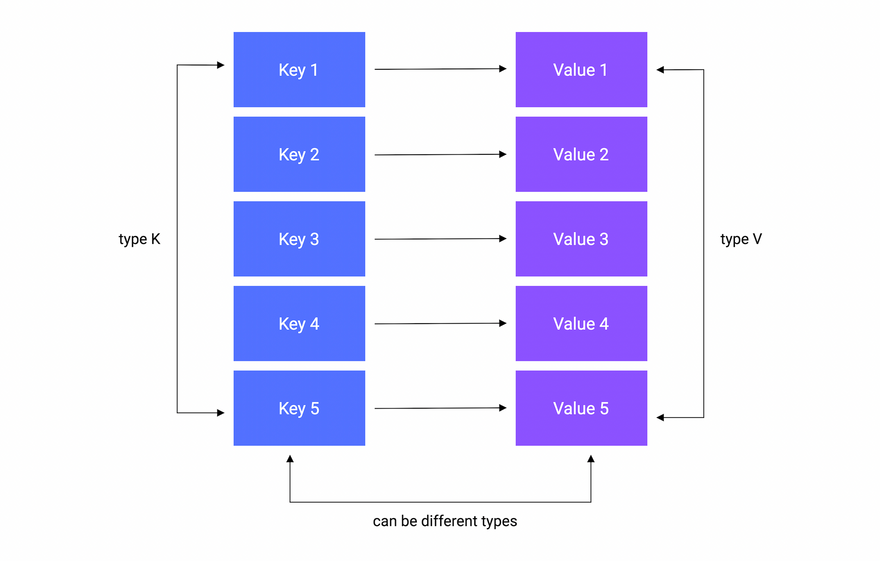Well, A map is an unordered collection of key-value pairs. It maps keys to values. The keys are unique within a map while the values may not be.

It is used for fast lookups, retrieval, and deletion of data based on keys. It is one of the most used data structures.

### Declaration

A map is declared using the following syntax

``````var m map[K]V
``````

Where `K` is the key type and `V` is the value type

For example, here's how we can declare a map of `string` keys to `int` values

``````func main() {
var m map[string]int

fmt.Println(m)
}
``````
``````\$ go run main.go
nil
``````

As we can see, the zero value of a map is `nil`.

A `nil`map has no keys. Moreover, any attempt to add keys to a `nil`map will result in a runtime error.

### Initialization

There are multiple ways to initialize a map.

make function

We can use the built-in `make` function, which allocates memory for referenced data types and initializes their underlying data structures.

``````func main() {
var m = make(map[string]int)

fmt.Println(m)
}
``````
``````\$ go run main.go
map[]
``````

map literal

Another way is using map literal.

``````func main() {
var m = map[string]int{
"a": 0,
"b": 1,
}

fmt.Println(m)
}
``````

Note that the last trailing comma is necessary

``````\$ go run main.go
map[a:0 b:1]
``````

As always, we can use our custom types as well

``````type User struct {
Name string
}

func main() {
var m = map[string]User{
"a": User{"Peter"},
"b": User{"Seth"},
}

fmt.Println(m)
}
``````

We can even remove the value type and Go will figure it out!

``````var m = map[string]User{
"a": {"Peter"},
"b": {"Seth"},
}
``````
``````\$ go run main.go
map[a:{Peter} b:{Seth}]
``````

Now, let's see how we can add a value to our map.

``````func main() {
var m = map[string]User{
"a": {"Peter"},
"b": {"Seth"},
}

m["c"] = User{"Steve"}

fmt.Println(m)
}
``````
``````\$ go run main.go
map[a:{Peter} b:{Seth} c:{Steve}]
``````

### Retrieve

We can also retrieve our values from the map using the key

``````...
c := m["c"]
fmt.Println("Key c:", c)
``````
``````\$ go run main.go
key c: {Steve}
``````

What if we use a key that is not present in the map?

``````...
d := m["d"]
fmt.Println("Key d:", d)
``````

Yes, you guessed it! we will get the zero value of the map's value type.

``````\$ go run main.go
Key c: {Steve}
Key d: {}
``````

### Exists

When you retrieve the value assigned to a given key, it returns an additional boolean value as well. The boolean variable will be `true` if the key exists, and `false` otherwise.

Let's try this in an example

``````...
c, ok := m["c"]
fmt.Println("Key c:", c, ok)

d, ok := m["d"]
fmt.Println("Key d:", d, ok)
``````
``````\$ go run main.go
Key c: {Steve} Present: true
Key d: {} Present: false
``````

### Updating

We can also update the value for a key by simply re-assigning a key

``````...
m["a"] = "Roger"
``````
``````\$ go run main.go
map[a:{Roger} b:{Seth} c:{Steve}]
``````

### Deleting

Or, we can delete the key using the built-in `delete` function.

Here's how the syntax looks

``````...
delete(m,
``````

The first argument is the map, and the second is the key we want to delete.

The `delete()` function doesn't return any value. Also, it doesn't do anything if the key doesn't exist in the map.

``````\$ go run main.go
map[a:{Roger} c:{Steve}]
``````

### Iteration

Similar to arrays or slices, we can iterate over maps with the `range` keyword

``````package main

import "fmt"

func main() {
var m = map[string]User{
"a": {"Peter"},
"b": {"Seth"},
}

m["c"] = User{"Steve"}

for key, value := range m {
fmt.Println("Key: %s, Value: %v", key, value)
}
}
``````
``````\$ go run main.go
Key: c, Value: {Steve}
Key: a, Value: {Peter}
Key: b, Value: {Seth}
``````

Note that, A map is an unordered collection, and therefore the iteration order of a map is not guaranteed to be the same every time we iterate over it.

### Properties

Lastly, let's talk about map properties.

Maps are reference types, which means when we assign a map to a new variable, they both refer to the same underlying data structure.

Therefore, changes done by one variable will be visible to the other.

``````package main

import "fmt"

type User struct {
Name string
}

func main() {
var m1 = map[string]User{
"a": {"Peter"},
"b": {"Seth"},
}

m2 := m1
m2["c"] = User{"Steve"}

fmt.Println(m1) // Output: map[a:{Peter} b:{Seth} c:{Steve}]
fmt.Println(m2) // Output: map[a:{Peter} b:{Seth} c:{Steve}]
}
``````

Well, this wraps up our discussion on maps, see you in the next tutorial!

# Interfaces

In this section, let's talk about the interfaces.

### What is an interface?

So, an interface in Go is an abstract type that is defined using a set of method signatures. The interface defines the behavior for similar types of objects.

Here, behavior is a key term that we will discuss shortly.

Let's take an example to understand this better.

One of the best real-world examples of interfaces is the power socket. Imagine that we need to connect different devices to the power socket.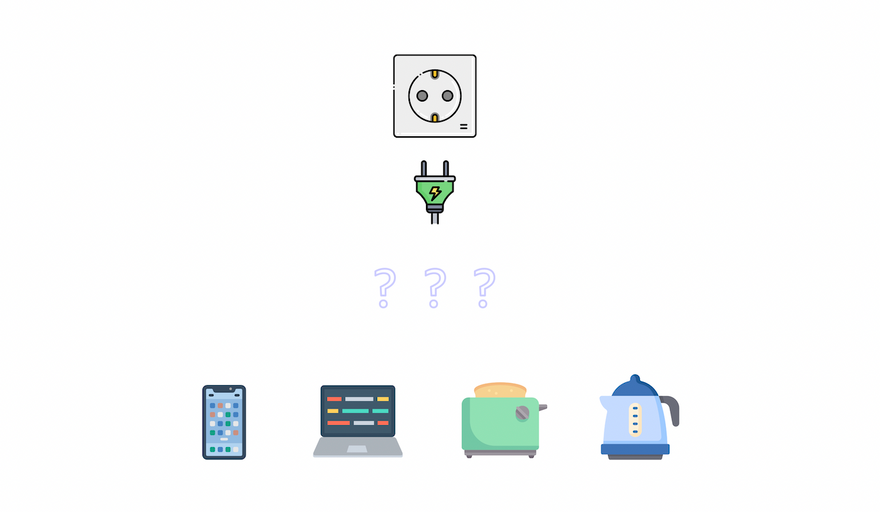Let's try to implement this. Here are the device types we will be using.

``````type mobile struct {
brand string
}

type laptop struct {
cpu string
}

type toaster struct {
amount int
}

type kettle struct {
quantity string
}

type socket struct{}
``````

Now let's define a `Draw` method on a type, let's say `mobile`. Here we will simply print the properties of the type.

``````func (m mobile) Draw(power int) {
fmt.Printf("%T -> brand: %s, power: %d", m, m.brand, power)
}
``````

Great, now we will define the `Plug` method on the `socket` type which accepts our `mobile` type as an argument.

``````func (socket) Plug(device mobile, power int) {
device.Draw(power)
}
``````

Let's try to “connect” or “plug in” the `mobile` type to our `socket` type in the `main` function

``````package main

import "fmt"

func main() {
m := mobile{"Apple"}

s := socket{}
s.Plug(m, 10)
}
``````

And if we run this we'll see the following

``````\$ go run main.go
main.mobile -> brand: Apple, power: 10
``````

This is interesting but let's say now we want to connect **our `laptop` type.

``````package main

import "fmt"

func main() {
m := mobile{"Apple"}
l := laptop{"Intel i9"}

s := socket{}

s.Plug(m, 10)
s.Plug(l, 50) // Error: cannot use l as mobile value in argument
}
``````

As we can see this will throw an error.

What should we do now? Define another method? Such as `PlugLaptop`?

Sure, but then every time we add a new device type we will need to add a new method to the socket type as well and that's not ideal.

This is where the `interface` comes in. Essentially we want to define a contract which in the future must be implemented.

We can simply define an interface such as `PowerDrawer` and use it in our `Plug` function to allow any device that satisfies the criteria, which is that the type must have a `Draw` method matching the signature that the interface requires.

And anyways the socket doesn't need to know anything about our device and can simply call the `Draw` method.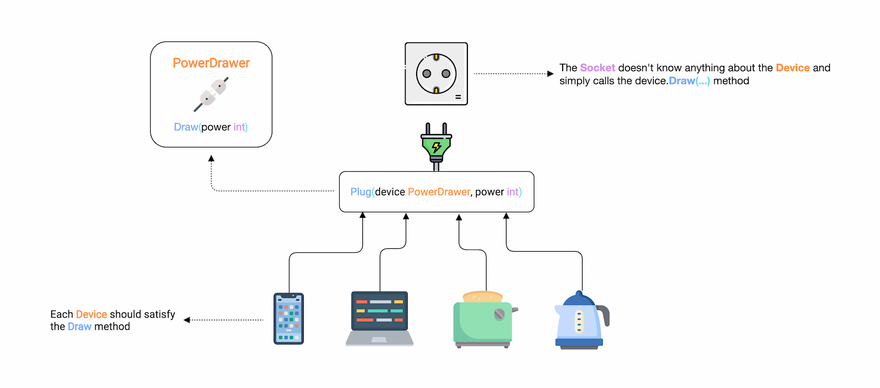Now let's try to implement our `PowerDrawer` interface. Here's how it will look.

The convention is to use “-er” as a suffix in the name. And as we discussed earlier, an interface should only describe the expected behavior. Which in our case is the `Draw` method.

``````type PowerDrawer interface {
Draw(power int)
}
``````

Now we need to update our `Plug` method to accept a device that implements the `PowerDrawer` interface as an argument.

``````func (socket) Plug(device PowerDrawer, power int) {
device.Draw(power)
}
``````

And to satisfy the interface, we can simply add `Draw` methods to all the device types.

``````type mobile struct {
brand string
}

func (m mobile) Draw(power int) {
fmt.Printf("%T -> brand: %s, power: %d\n", m, m.brand, power)
}

type laptop struct {
cpu string
}

func (l laptop) Draw(power int) {
fmt.Printf("%T -> cpu: %s, power: %d\n", l, l.cpu, power)
}

type toaster struct {
amount int
}

func (t toaster) Draw(power int) {
fmt.Printf("%T -> amount: %d, power: %d\n", t, t.amount, power)
}

type kettle struct {
quantity string
}

func (k kettle) Draw(power int) {
fmt.Printf("%T -> quantity: %s, power: %d\n", k, k.quantity, power)
}
``````

Now we can connect all our devices to the socket with the help of our interface!

``````func main() {
m := mobile{"Apple"}
l := laptop{"Intel i9"}
t := toaster{4}
k := kettle{"50%"}

s := socket{}

s.Plug(m, 10)
s.Plug(l, 50)
s.Plug(t, 30)
s.Plug(k, 25)
}
``````

And just as we expected, it works.

``````\$ go run main.go
main.mobile -> brand: Apple, power: 10
main.laptop -> cpu: Intel i9, power: 50
main.toaster -> amount: 4, power: 30
main.kettle -> quantity: Half Empty, power: 25
``````

But why is this considered such a powerful concept?

Well, an interface can help us decouple our types. For example, because we have the interface, we don't need to update our `socket` implementation. We can just define a new device type with a `Draw` method.

Unlike other languages, Go Interfaces are implemented implicitly so we don't need something like an `implements` keyword. This means, that a type satisfies an interface automatically when it has "all the methods” of the interface.

### Empty Interface

Next, let's talk about the empty interface. An empty interface can take on a value of any type.

Here's how we declare it.

``````var x interface{}
``````

But why do we need it?

Empty interfaces can be used to handle values of unknown type.

Some examples are:

• Reading heterogeneous data from an API
• Variables of an unknown type, like in the `fmt.Prinln` function

To use a value of type empty `interface{}`, we can use type assertion or a type switch to determine the type of the value.

### Type Assertion

A type assertion provides access to an interface value's underlying concrete value.

For example

``````func main() {
var i interface{} = "hello"

s := i.(string)
fmt.Println(s)
}
``````

This statement asserts that the interface value holds a concrete type and assigns the underlying type value to the variable.

We can also test whether an interface value holds a specific type.

A type assertion can return two values:

• The first one is the underlying value
• The second is a boolean value that reports whether the assertion succeeded.
``````s, ok := i.(string)
fmt.Println(s, ok)
``````

This can help us test whether an interface value holds a specific type or not.

In a way, this is similar to how we read values from a map.

And If this is not the case then, `ok` will be false and the value will be the zero value of the type, and no panic will occur.

``````f, ok := i.(float64)
fmt.Println(f, ok)
``````

But If the interface does not hold the type, the statement will trigger a panic.

``````f = i.(float64)
fmt.Println(f) // Panic!
``````
``````\$ go run main.go
hello
hello true
0 false
panic: interface conversion: interface {} is string, not float64
``````

### Type Switch

Here, a `switch` statement can be used to determine the type of a variable of type empty `interface{}`.

``````var t interface{}
t = "hello"

switch t := t.(type) {
case string:
fmt.Printf("string: %s\n", t)
case bool:
fmt.Printf("boolean: %v\n", t)
case int:
fmt.Printf("integer: %d\n", t)
default:
fmt.Printf("unexpected: %T\n", t)
}
``````

And if run this, we can verify that we have a `string` type

``````\$ go run main.go
string: hello
``````

### Properties

Let's discuss some properties of interfaces.

Zero value

The zero value of an interface is `nil`

``````package main

import "fmt"

type MyInterface interface {
Method()
}

func main() {
var i MyInterface

fmt.Println(i) // Output: <nil>
}
``````

Embedding

We can embed interfaces like structs.

For example

``````type interface1 interface {
Method1()
}

type interface2 interface {
Method2()
}

type interface3 interface {
interface1
interface2
}
``````

Values

Interface values are comparable

``````package main

import "fmt"

type MyInterface interface {
Method()
}

type MyType struct{}

func (MyType) Method() {}

func main() {
t := MyType{}
var i MyInterface = MyType{}

fmt.Println(t == i)
}
``````

Interface Values

Under the hood, an interface value can be thought of as a tuple consisting of a value and a concrete type.

``````package main

import "fmt"

type MyInterface interface {
Method()
}

type MyType struct {
property int
}

func (MyType) Method() {}

func main() {
var i MyInterface

i = MyType{10}

fmt.Printf("(%v, %T)\n", i, i) // Output: ({10}, main.MyType)
}
``````

With that, we covered interfaces in Go.

It's a really powerful feature but remember “Bigger the interface, the weaker the abstraction” - Rob Pike.

# Errors

In this tutorial, let's talk about error handling.

Notice I said errors and not exceptions as there is no exception handling in Go.

Instead, we can just return a built-in `error` type which is an interface type.

``````type error interface {
Error() string
}
``````

We will circle back to this shortly. First, let's try to understand the basics.

So, Let's declare a simple `Divide` function which as the name suggests, will divide integer `a` by `b`

``````func Divide(a, b int) int {
return a/b
}
``````

Great. Now, we want to return an error, let's say to prevent the division by zero. This brings us to error construction.

## Constructing Errors

There are multiple ways to do this but we will look at the two most common one.

### `errors` package

The first is by using the `New` function provided by the `errors` package.

``````package main

import "errors"

func main() {}

func Divide(a, b int) (int, error) {
if b == 0 {
return 0, errors.New("cannot divide by zero")
}

return a/b, nil
}
``````

Notice, how we return error with the result. And if there is no error we simply return `nil` as it is the zero value of an error because after all, it's an interface.

But how do we handle it? So, for that let's call the `Divide` function in our `main` function.

``````package main

import (
"errors"
"fmt"
)

func main() {
result, err := Divide(4, 0)

if err != nil {
fmt.Println(err)
// Do something with the error
return
}

fmt.Println(result)
// Use the result
}

func Divide(a, b int) (int, error) {...}
``````
``````\$ go run main.go
cannot divide by zero
``````

As you can see, we simply check if the error is `nil` and build our logic accordingly. This is considered quite idiomatic in Go and you will see this being used a lot.

Another way to construct our errors is by using the `fmt.Errorf` function.

This function is similar to `fmt.Sprintf` and lets us format our error but instead of returning a string, it returns an error.

It is often used to add some context or detail to our errors.

``````...
func Divide(a, b int) (int, error) {
if b == 0 {
return 0, fmt.Errorf("cannot divide %d by zero", a)
}

return a/b, nil
}
``````

And it should work similarly

``````\$ go run main.go
cannot divide 4 by zero
``````

### Sentinel Errors

Another important technique in Go is defining expected Errors so they can be checked explicitly in other parts of the code. These are sometimes referred to as sentinel errors.

``````package main

import (
"errors"
"fmt"
)

var ErrDivideByZero = errors.New("cannot divide by zero")

func main() {...}

func Divide(a, b int) (int, error) {
if b == 0 {
return 0, ErrDivideByZero
}

return a/b, nil
}
``````

In Go, it is considered conventional to prefix the variable with `Err`.

For example, `ErrNotFound`

But what's the point?

So, this becomes useful when we need to execute a different branch of code if a certain kind of error is encountered.

For example, now we can check explicitly which error occurred using the `errors.Is` function

``````package main

import (
"errors"
"fmt"
)

func main() {
result, err := Divide(4, 0)

if err != nil {
switch {
case errors.Is(err, ErrDivideByZero):
fmt.Println(err)
// Do something with the error
default:
fmt.Println("no idea!")
}

return
}

fmt.Println(result)
// Use the result
}

func Divide(a, b int) (int, error) {...}
``````
``````\$ go run main.go
cannot divide by zero
``````

## Custom Errors

This strategy covers most of the error handling use cases. But sometimes we need additional functionalities such as dynamic values inside of our errors.

Earlier we saw that `error` is just an interface. So basically, anything can be an `error` as long as it implements the `Error()` method which returns an error message as a string.

So let's define our custom `DivisionError` struct which will contain an error code and a message.

``````package main

import (
"errors"
"fmt"
)

type DivisionError struct {
Code int
Msg  string
}

func (d DivisionError) Error() string {
return fmt.Sprintf("code %d: %s", d.Code, d.Msg)
}

func main() {...}

func Divide(a, b int) (int, error) {
if b == 0 {
return 0, DivisionError{
Code: 2000,
Msg:  "cannot divide by zero",
}
}

return a/b, nil
}
``````

Here, we will use `errors.As` instead of `errors.Is` function, to convert the error to the correct type.

``````func main() {
result, err := Divide(4, 0)

if err != nil {
var divErr DivisionError

switch {
case errors.As(err, &divErr):
fmt.Println(divErr)
// Do something with the error
default:
fmt.Println("no idea!")
}

return
}

fmt.Println(result)
// Use the result
}

func Divide(a, b int) (int, error) {...}
``````
``````\$ go run man.go
code 2000: cannot divide by zero
``````

But what's the difference between `errors.Is` and `errors.As`?

The difference is that this function checks whether the error has a specific type, unlike the `Is()`, which examines if it is a particular error object.

We can also use type assertions, but it's not preferred

``````func main() {
result, err := Divide(4, 0)

if e, ok := err.(DivisionError); ok {
fmt.Println(e.Code, e.Msg) // Output: 2000 cannot divide by zero
return
}

fmt.Println(result)
}
``````

Lastly, I will say that error handling in Go is quite different compared to the traditional `try/catch` idiom in other languages. But it is very powerful as it encourages the developer to actually handle the error in an explicit way, which improves readability as well.

I hope this tutorial helped you learn about errors in Go and how to handle them. I'll see you in the next one!

# Panic and Recover

So earlier we learned that the idiomatic way of handling abnormal conditions in a Go program is using errors. While errors are sufficient for most cases, there are some situations where the program cannot continue.

In those cases, we can use the built-in `panic` function.

``````func panic(interface{})
``````

The panic is a built-in function that stops the normal execution of the current `goroutine`. When a function calls `panic`, the normal execution of the function stops immediately and the control is returned back to the caller. This is repeated until the program exits with the panic message and stack trace.

Note: We will discuss `goroutines` later in the course

So let's see how we can use the `panic` function

``````package main

func main() {
WillPanic()
}

func WillPanic() {
panic("Woah")
}
``````

And if we run this, we can see `panic` in action

``````\$ go run main.go
panic: Woah

goroutine 1 [running]:
main.WillPanic(...)
.../main.go:8
main.main()
.../main.go:4 +0x38
exit status 2
``````

As expected, our program printed the panic message, followed by the stack trace, and then it was terminated.

So the question is, what to do when an unexpected panic happens?

Well, It is possible to regain control of a panicking program using the built-in `recover` function along with the `defer` keyword.

``````func recover() interface{}
``````

Let's try an example by creating a `handlePanic` function. And then we can all it using `defer`

``````package main

import "fmt"

func main() {
WillPanic()
}

func handlePanic() {
data := recover()
fmt.Println("Recovered:", data)
}

func WillPanic() {
defer handlePanic()

panic("Woah")
}
``````
``````\$ go run main.go
Recovered: Woah
``````

As we can see, our panic was recovered and now our program can continue execution.

Lastly, I will mention that `panic` and `recover` can be considered similar to the `try/catch` idiom in other languages. One important factor is that we should avoid panic and recover and use errors when possible.

If so then this brings us to the question, when should we use `panic`?

There are two valid use cases for `panic`:

• An unrecoverable error

Which can be a situation where the program cannot simply continue its execution.

For example, reading a configuration file which is important to start the program, as there is nothing else to do if the file read itself fails.

• Developer error

This is the most common situation.

For example, dereferencing a pointer when the value is `nil` will cause a panic.

I hope this tutorial helped you understand how to use `panic` and `recover` in Go. I'll see you in the next one.

# Testing

In this tutorial, we will talk about testing in Go. So, let's start using a simple example.

We have created a `math` package that contains an `Add` function which as the name suggests adds two integers.

``````package math

func Add(a, b int) int {
return a + b
}
``````

It's being used in our `main` package like this

``````package main

import (
"example/math"
"fmt"
)

func main() {
fmt.Println(result)
}

``````

And, if we run this, we should see the result

``````\$ go run main.go
4
``````

Now, we want to test our `Add` function. So, in Go, we declare tests files with `_test` suffix in the file name. So for our `add.go`, we will create a test as `add_test.go`

Our project structure should look like this.

``````.
├── go.mod
├── main.go
└── math
``````

We will start by using a `math_test` package, and import the `testing` package from the standard library. That's right! testing is built into Go, unlike many other languages.

But wait...why do we need to use `math_test` as our package, can't we just use the same `math` package?

Well yes, we can write our test in the same package if we wanted but I personally think doing this in a separate package helps us write tests in a more decoupled way.

Now we can create our `TestAdd` function. It will take an argument of type `testing.T` which will provide us with helpful methods.

``````package math_test

import "testing"

``````

Before we add any testing logic, let's try to run it. But this time we cannot use `go run` command, instead, we will use the `go test` command.

``````\$ go test ./math
ok      example/math 0.429s
``````

Here, we will our package name which is `math` but we can also use the relative path `./...` to test all packages.

``````\$ go test ./...
?       example [no test files]
ok      example/math 0.348s
``````

And if Go doesn't find any test in a package it will let us know.

Perfect, let's write some test code. To do this, we will check our result with an expected value and if they do not match, we can use the `t.Fail` method to fail the test.

``````package math_test

import "testing"

expected := 2

if got != expected {
t.Fail()
}
}
``````

Great! our test seems to have passed.

``````\$ go test math
ok      example/math    0.412s
``````

Let's also see what happens if we fail the test, so for that, we can change our expected result

``````package math_test

import "testing"

expected := 3

if got != expected {
t.Fail()
}
}
``````
``````\$ go test ./math
ok      example/math    (cached)
``````

If you see this, don't worry. For optimization, our tests are cached. We can use the `go clean` command to clear our cache and then re-run the test.

``````\$ go clean -testcache
\$ go test ./math
FAIL
FAIL    example/math    0.354s
FAIL
``````

So this is what a test failure will look like.

### Table driven tests

This brings us to table-driven tests. But what exactly are they?

So earlier we had function arguments and expected variables which we compared to determine if our tests passed or fail. But what if we defined all that in a slice and iterate over that? This will make our tests a little bit more flexible and help us run multiple cases easily.

Don't worry, we will learn this by example. So we will start by defining our `addTestCase` struct.

``````package math_test

import (
"example/math"
"testing"
)

a, b, expected int
}

{1, 1, 3},
{25, 25, 50},
{2, 1, 3},
{1, 10, 11},
}

for _, tc := range testCases {

if got != tc.expected {
t.Errorf("Expected %d but got %d", tc.expected, got)
}
}
}
``````

Notice, how we declared `addTestCase` with a lower case. That's right we don't want to export it as it's not useful outside our testing logic.

Let's run our test

``````\$ go run main.go
add_test.go:25: Expected 3 but got 2
FAIL
FAIL    example/math    0.334s
FAIL
``````

Seems like our tests broke, let's fix them by updating our test cases.

``````var testCases = []addTestCase{
{1, 1, 2},
{25, 25, 50},
{2, 1, 3},
{1, 10, 11},
}
``````

Perfect, it's working!

``````\$ go run main.go
ok      example/math    0.589s
``````

### Code coverage

Finally, let's talk about code coverage. When writing tests, it is often important to know how much of your actual code the tests cover. This is generally referred to as code coverage.

To calculate and export the coverage for our test, we can simply use the `-coverprofile` argument with the `go test` command.

``````\$ go test ./math -coverprofile=coverage.out
ok      example/math    0.385s  coverage: 100.0% of statements
``````

Seems like we have great coverage. Let's also check the report using the `go tool cover` command which gives us a detailed report.

``````\$ go tool cover -html=coverage.out
``````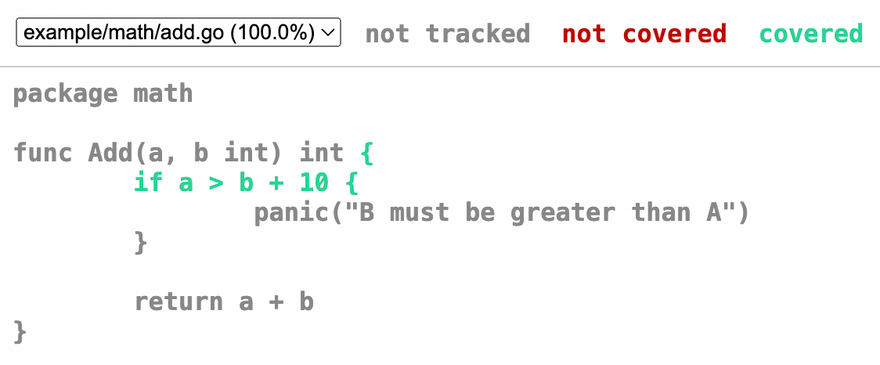As we can see this is a much more readable format. And best of all, it is built right into standard tooling.

### Fuzz testing

Lastly, let's look at fuzz testing which was introduced in Go version 1.18.

Fuzzing is a type of automated testing that continuously manipulates inputs to a program to find bugs.

Go fuzzing uses coverage guidance to intelligently walk through the code being fuzzed to find and report failures to the user.

Since it can reach edge cases that humans often miss, fuzz testing can be particularly valuable for finding bugs and security exploits.

Let's try an example,

``````func FuzzTestAdd(f *testing.F) {
f.Fuzz(func(t *testing.T, a, b int) {
})
}
``````

If we run this, we'll see that it'll automatically create test cases. Because our `Add` function is quite simple, tests will pass.

``````\$ go test -fuzz FuzzTestAdd example/math
fuzz: elapsed: 0s, gathering baseline coverage: 0/192 completed
fuzz: elapsed: 0s, gathering baseline coverage: 192/192 completed, now fuzzing with 8 workers
fuzz: elapsed: 3s, execs: 325017 (108336/sec), new interesting: 11 (total: 202)
fuzz: elapsed: 6s, execs: 680218 (118402/sec), new interesting: 12 (total: 203)
fuzz: elapsed: 9s, execs: 1039901 (119895/sec), new interesting: 19 (total: 210)
fuzz: elapsed: 12s, execs: 1386684 (115594/sec), new interesting: 21 (total: 212)
PASS
ok      foo 12.692s
``````

But if we update our `Add` function with a random edge case such where the program will panic if `b + 10` is greater than `a`.

``````func Add(a, b int) int {
if a > b + 10 {
panic("B must be greater than A")
}

return a + b
}
``````

And if we re-run the test, this edge case will be caught by fuzz testing.

``````\$ go test -fuzz FuzzTestAdd example/math
warning: starting with empty corpus
fuzz: elapsed: 0s, execs: 0 (0/sec), new interesting: 0 (total: 0)
fuzz: elapsed: 0s, execs: 1 (25/sec), new interesting: 0 (total: 0)
testing.go:1349: panic: B is greater than A
``````

I think this is a really cool feature of Go 1.18. You can learn more about fuzz testing from the official Go blog.

Perfect, so this is pretty much it for this tutorial. I'll see you in the next one!

# Generics

In this section, we will learn about Generics which is a much awaited feature that was released with Go version 1.18

### What are Generics?

Generics means parameterized types. Put simply, generics allow programmers to write code where the type can be specified later because the type isn't immediately relevant

Let's take an example to understand this better.

For our example, we have simple sum functions for different types such as `int`, `float64`, and `string`. Since method overriding is not allowed in Go we usually have to create new functions.

``````package main

import "fmt"

func sumInt(a, b int) int {
return a + b
}

func sumFloat(a, b float64) float64 {
return a + b
}

func sumString(a, b string) string {
return a + b
}

func main() {
fmt.Println(sumInt(1, 2))
fmt.Println(sumFloat(4.0, 2.0))
fmt.Println(sumString("a", "b"))
}
``````

As we can see, apart from the types, these functions are pretty similar.

Let's see how we can define a generic function.

``````func fnName[T constraint]() {
...
}
``````

Here, `T` is our type parameter and **`constraint` will be the interface that allows any type **implementing the interface**.

I know I know, this is confusing. So let's start building our generic `sum` function.

Here we will use `T` as our type parameter with an empty `interface{}` as our constraint.

``````func sum[T interface{}](a, b T) T {
fmt.Println(a, b)
}
``````

Also, starting with Go 1.18 we can use `any` which is pretty much equivalent to the empty interface.

``````func sum[T any](a, b T) T {
fmt.Println(a, b)
}
``````

With type parameters comes the need to pass type arguments, which can make our code verbose.

``````sum[int](1, 2) // explicit type argument
sum[float64](4.0, 2.0)
sum[string]("a", "b")
``````

Luckily Go 1.18 comes with type inference which helps us to write code that calls generic functions without explicit types.

``````sum(1, 2)
sum(4.0, 2.0)
sum("a", "b")
``````

Let's run this and see if it works

``````\$ go run main.go
1 2
4 2
a b
``````

Now let's update the `sum` function to add our variables.

``````func sum[T any](a, b T) T {
return a + b
}
``````
``````fmt.Println(sum(1, 2))
fmt.Println(sum(4.0, 2.0))
fmt.Println(sum("a", "b"))
``````

But now if we run this, we will get an error that operator `+` is not defined in the constraint.

``````\$ go run main.go
./main.go:6:9: invalid operation: operator + not defined on a (variable of type T constrained by any)
``````

While constraint of type `any` generally works it does not support operators.

So let's define our own custom constraint using an interface. Our interface should define a type set containing `int`, `float`, and `string`.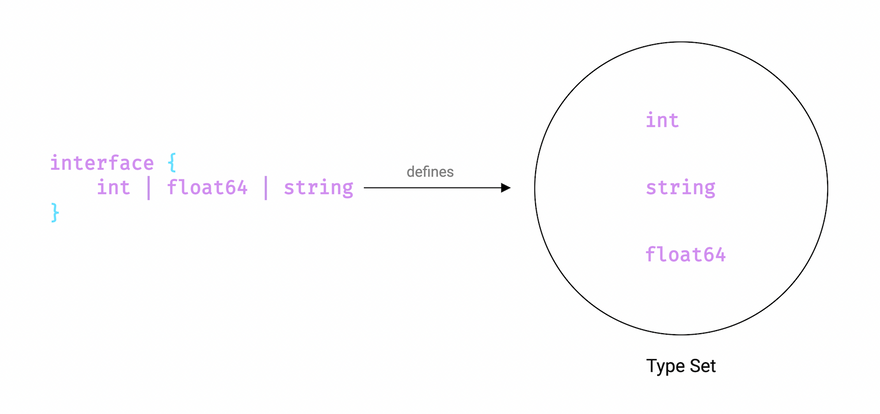Here's how our `SumConstraint` interface looks

``````type SumConstraint interface {
int | float64 | string
}

func sum[T SumConstraint](a, b T) T {
return a + b
}

func main() {
fmt.Println(sum(1, 2))
fmt.Println(sum(4.0, 2.0))
fmt.Println(sum("a", "b"))
}
``````

And this should work as expected

``````\$ go run main.go
3
6
ab
``````

We can also use the `constraints` package which defines a set of useful constraints to be used with type parameters.For that, we will need to install the `constraints` package

``````\$ go get golang.org/x/exp/constraints
``````
``````import (
"fmt"

"golang.org/x/exp/constraints"
)

func sum[T constraints.Ordered](a, b T) T {
return a + b
}

func main() {
fmt.Println(sum(1, 2))
fmt.Println(sum(4.0, 2.0))
fmt.Println(sum("a", "b"))
}
``````

Here we are using the `Ordered` constraint.

``````type Ordered interface {
Integer | Float | ~string
}
``````

`~` is a new token added to Go and the expression `~string` means the set of all types whose underlying type is `string`

And it still works as expected

``````\$ go run main.go
3
6
ab
``````

Generics is an amazing feature because it permits writing abstract functions that can drastically reduce code duplication in certain cases.

### When to use generics

So, when to use generics? We can take the following use cases as an example

• Functions that operate on arrays, slices, maps, and channels
• General purpose data structures like stack or linked list
• To reduce code duplication

Lastly, I will add that while generics are a great addition to the language They should be used sparingly.

And, it is advised to start simple and only write generic code once we have written very similar code at least 2 or 3 times.

Perfect, this wraps us our discussion on generics. I'll see you in the next tutorial!

# Concurrency

In this lesson, we will learn about concurrency which is one of the most powerful features of Go.

So let's start by asking What is “concurrency”?

## What is Concurrency

Concurrency, by definition, is the ability to break down a computer program or algorithm into individual parts, which can be executed independently.

The final outcome of a concurrent program is the same as that of a program that has been executed sequentially.

Using concurrency, we are able to achieve the same results in lesser time, thus increasing the overall performance and efficiency of our programs.

## Concurrency vs Parallelism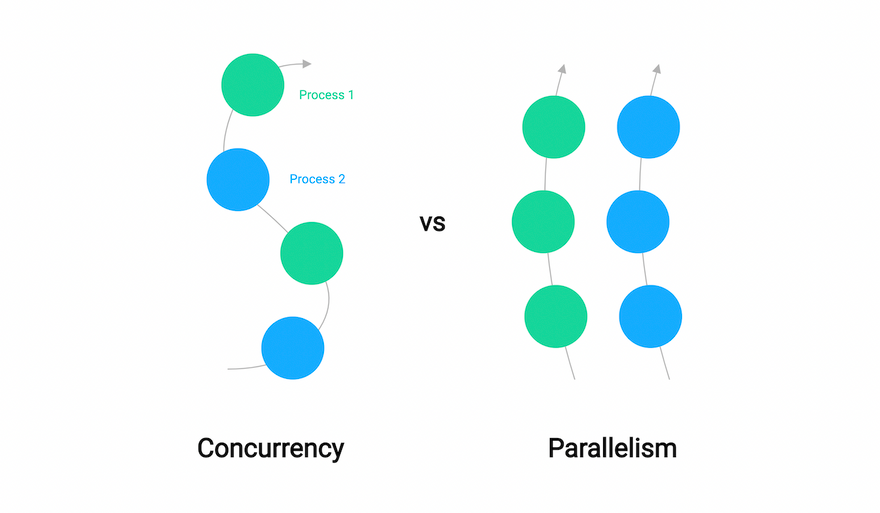A lot of people confuse concurrency with parallelism because they both somewhat imply executing code simultaneously but they are two completely different concepts.

Concurrency is the task of running and managing multiple computations at the same time. While parallelism is the task of running multiple computations simultaneously.

A simple quote from Rob Pike pretty much sums it up.

“Concurrency is about dealing with lots of things at once. Parallelism is about doing lots of things at once”

But concurrency in Go is more than just syntax. In order to harness the power of Go, we need to first understand how Go approaches concurrent execution of code. Go relies on a concurrency model called CSP (Communicating Sequential Processes).

## Communicating Sequential Processes (CSP)

Communicating Sequential Processes (CSP) is a model put forth by Tony Hoare in 1978 which describes interactions between concurrent processes. It made a breakthrough in Computer Science, especially in the field of concurrency.

Languages like Go and Erlang have been highly inspired by the concept of communicating sequential processes (CSP).

Concurrency is hard but CSP allows us to give a better structure to our concurrent code and provides a model for thinking about concurrency in a way that makes it a little easier. Here, processes are independent and they communicate by sharing channels between them.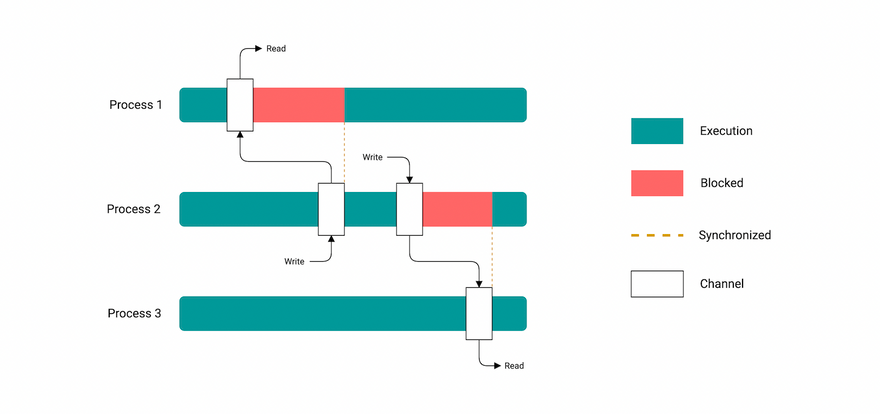We'll learn how Golang implements it using goroutines and channels later in the course.

## Basic Concepts

Now let's get familiar with some basic concurrency concepts

### Data Race

A data race occurs when processes have to access the same variable concur­rently.

For example, one process reads while another simultaneously writes to the exact same variable.

### Race Conditions

A race condition occurs when the timing or order of events affects the correctness of a piece of code.

A deadlock occurs when all processes are blocked while waiting for each other and the program cannot proceed further.

Coffman Conditions

There are four conditions, known as the Coffman conditions, all of them must be satisfied for a deadlock to occur.

• Mutual Exclusion

A concurrent process holds at least one resource at any one time making it non-sharable.

In the diagram below, there is a single instance of Resource 1 and it is held by Process 1 only.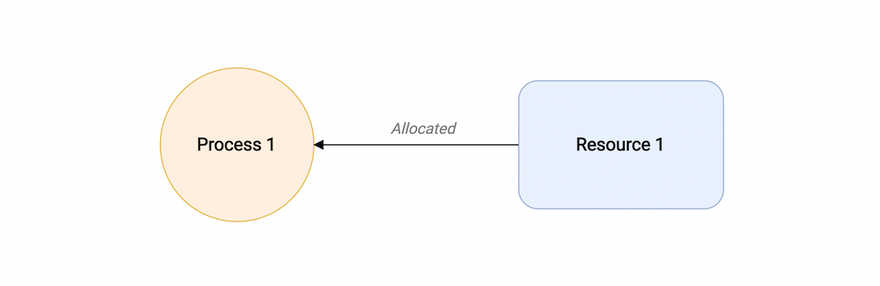• Hold and wait

A concurrent process holds a resource and is waiting for an additional resource.

In the diagram given below, Process 2 holds Resource 2 and Resource 3 and is requesting the Resource 1 which is held by Process 1.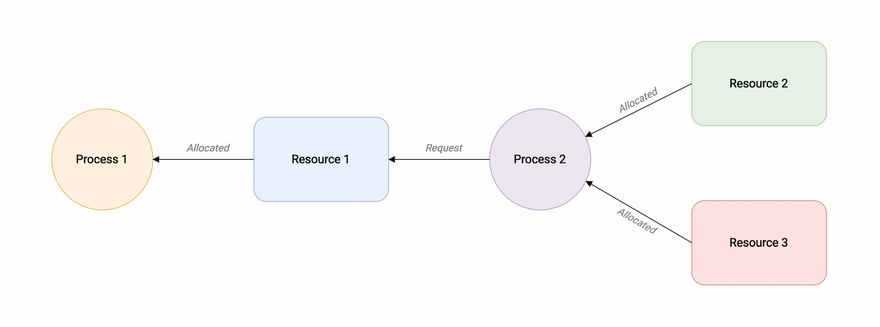• No preemption

A resource held by a concurrent process cannot be taken away by the system. It can only be freed by the process holding it.

In the diagram below, Process 2 cannot preempt Resource 1 from Process 1. It will only be released when Process 1 relinquishes it voluntarily after its execution is complete.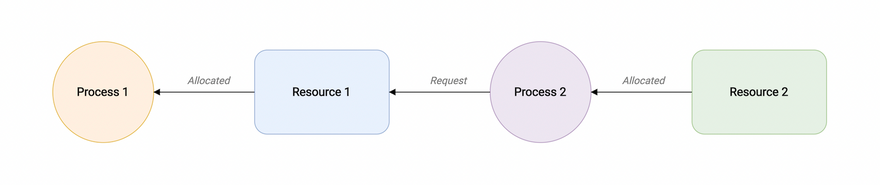• Circular wait

A process is waiting for the resource held by the second process, which is waiting for the resource held by the third process, and so on, till the last process is waiting for a resource held by the first process. Hence, forming a circular chain.

In the diagram below, Process 1 is allocated Resource2 and it is requesting Resource 1. Similarly, Process 2 is allocated Resource 1 and it is requesting Resource 2. This forms a circular wait loop.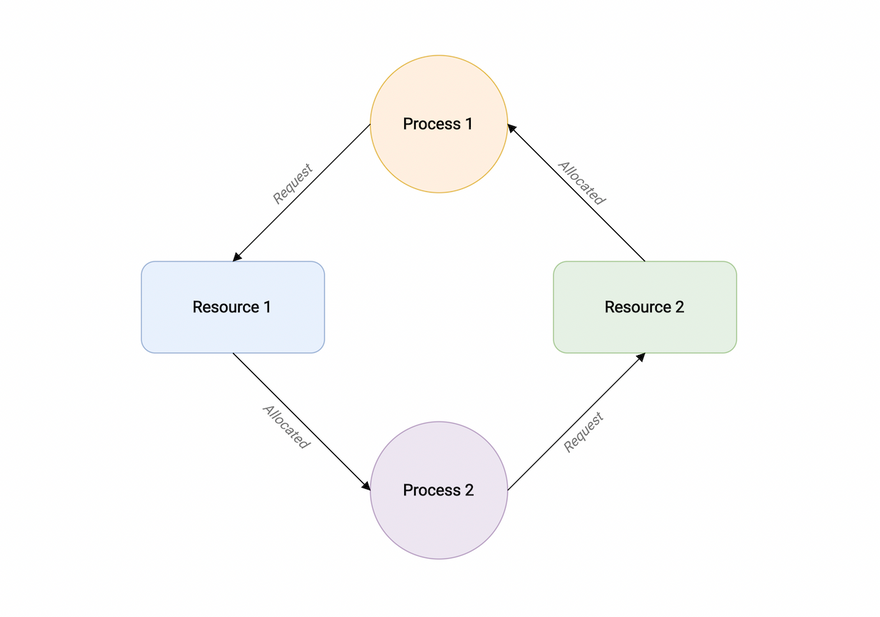### Starvation

Starvation happens when a process is deprived of necessary resources and is unable to complete its function.

Starvation can happen because of deadlocks or inefficient scheduling algorithms for processes. In order to solve starvation, we need to employ better resource-allotment algorithms that make sure that every process gets its fair share of resources.

This wraps up our discussion on the basics of concurrency in Go. I'll see you in the next lesson where we will learn about channels and goroutines.

# Goroutines

In this lesson, we will learn about Goroutines.

But before we start our discussion, I wanted to share an important Go proverb.

“Don't communicate by sharing memory, share memory by communicating.” - Rob Pike

## What is a goroutine?

A goroutine is a lightweight thread of execution that is managed by the Go runtime and essentially let us write asynchronous code in a synchronous manner.

It is important to know that they are not actual OS threads and the main function itself runs as a goroutine.

A single thread may run thousands of goroutines in them by using the Go runtime scheduler which uses cooperative scheduling. This implies that if the current goroutine is blocked or has been completed, the scheduler will move the other goroutines to another OS thread. Hence, we achieve efficiency in scheduling where no routine is blocked forever.

We can turn any function into a goroutine by simply using the go keyword

``````go fn(x, y, z)
``````

Before we write any code, it is important to briefly discuss the fork-join model.

## Fork-Join Model

Go uses the idea of the fork-join model of concurrency behind goroutines. The fork-join model essentially implies that a child process splits from its parent process to run concurrently with the parent process. After completing its execution, the child process merges back into the parent process. The point where it joins back is called the join point.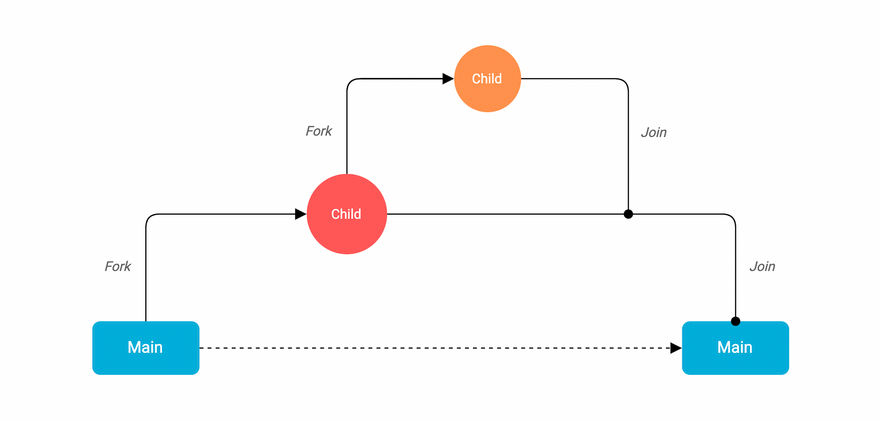Now let's write some code and create our own goroutine

``````package main

import "fmt"

func speak(arg string) {
fmt.Println(arg)
}

func main() {
go speak("Hello World")
}
``````

Here the `speak` function call is prefixed with the `go` keyword. This will allow it to run as a separate goroutine. And that's it, we just created our first goroutine. It's that simple!

Great, let's run this

``````\$ go run main.go

``````

Interesting, it seems like our program did not run completely as it's missing some output. This is because our main goroutine exited and did not wait for the goroutine that we created.

What if we make our program wait using the `time.Sleep` function?

``````func main() {
...
time.Sleep(1 * time.Second)
}
``````

And now if we run this

``````\$ go run main.go
Hello World
``````

There we go, we can see our complete output now.

Okay so this works but it's not ideal. So how do we improve this?

Well, the most tricky part about using goroutines is knowing when they will stop. It is important to know that goroutines run in the same address space, so access to shared memory must be synchronized.

This brings us to channels, which we will discuss in the next.

# Channels

In this lesson, we will learn about Channels.

### So what are channels?

Well, simply defined.

A channel is a communications pipe between goroutines. Things go in one end and come out another in the same order until the channel is closed.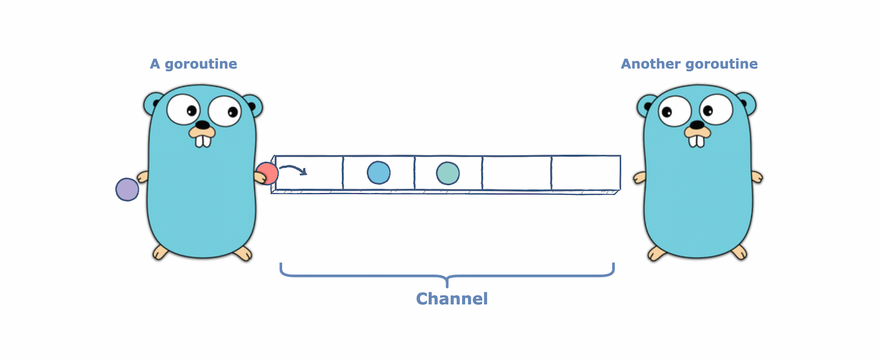As we learned earlier, channels in Go are based on Communicating Sequential Processes (CSP).

### Creating a channel

Now that we understand what channels are, let's see how we can declare them

``````var ch chan T
``````

Here, We prefix our type `T` which is the data type of the value we want to send and receive with the keyword `chan` which stands for a channel.

Let's try printing the value of our channel `c` of type `string`.

``````func main() {
var ch chan string

fmt.Println(c)
}
``````
``````\$ go run main.go
<nil>
``````

As we can see, the zero value of a channel is `nil` and if we try to send data over the channel our program will panic.

So similar to slices we can initialize our channel using the built-in `make` function.

``````func main() {
ch := make(chan string)

fmt.Println(c)
}
``````

And if we run this we can see our channel was initialized.

``````\$ go run main.go
0x1400010e060
``````

### Sending and Receiving data

Now that we have a basic understanding of channels, let us implement our earlier example using channels to learn how we can use them to communicate between our goroutines.

``````package main

import "fmt"

func speak(arg string, ch chan string) {
ch <- arg // Send
}

func main() {
ch := make(chan string)

go speak("Hello World", ch)

fmt.Println(data)
}
``````

Notice how we can send data using the `channel<-data` and receive data using the `data := <-channel` syntax.

And if we run this

``````\$ go run main.go
Hello World
``````

Perfect, our program ran as we expected.

### Buffered Channels

We also have buffered channels that accept a limited number of values without a corresponding receiver for those values.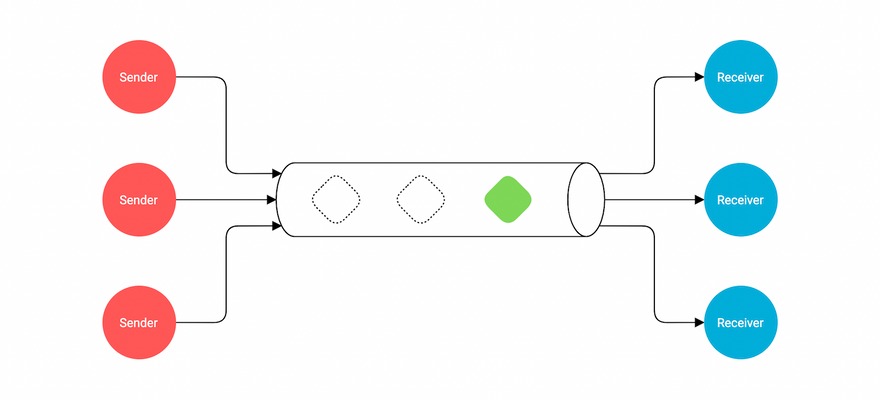This buffer length or capacity can be specified using the second argument to the `make` function.

``````func main() {
ch := make(chan string, 2)

go speak("Hello World", ch)
go speak("Hi again", ch)

data1 := <-ch
fmt.Println(data1)

data2 := <-ch
fmt.Println(data2)
}
``````

Because this channel is buffered, we can send these values into the channel without a corresponding concurrent receive.

By default a channel is unbuffered and has a capacity of 0, hence we omit the second argument to the `make` function.

Next, we have directional channels.

### Directional channels

When using channels as function parameters, we can specify if a channel is meant to only send or receive values. This increases the type-safety of our program as by default a channel can both send and receive values.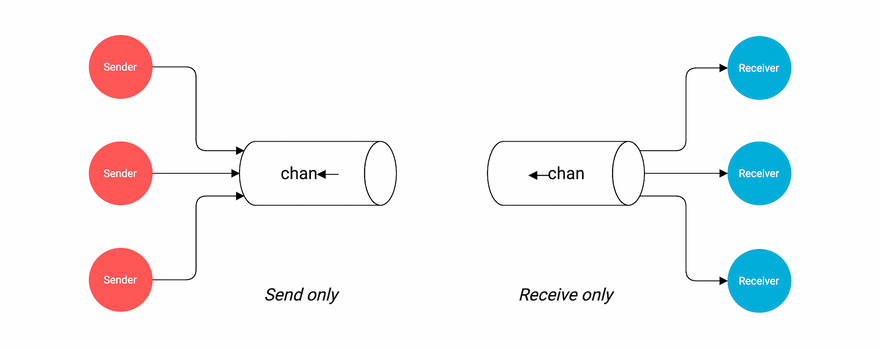In our example, we can update our `speak` function's second argument such that it can only send a value.

``````func speak(arg string, ch chan<- string) {
ch <- arg // Send Only
}
``````

Here, `chan<-` can only be used for sending values and will panic if we try to receive values.

### Closing channels

Also, just like any other resource, once we're done with our channel we need to close it. This can be achieved using the built-in `close` function.

Here, we can just pass our channel to the `close` function.

``````func main() {
ch := make(chan string, 2)

go speak("Hello World", ch)
go speak("Hi again", ch)

data1 := <-ch
fmt.Println(data1)

data2 := <-ch
fmt.Println(data2)

close(ch)
}
``````

Optionally, receivers can test whether a channel has been closed by assigning a second parameter to the receive expression.

``````func main() {
ch := make(chan string, 2)

go speak("Hello World", ch)
go speak("Hi again", ch)

data1 := <-ch
fmt.Println(data1)

data2, ok := <-ch
fmt.Println(data2, ok)

close(ch)
}
``````

if `ok` is `false` then there are no more values to receive and the channel is closed.

In a way, this is similar to how we check if a key exists or not in a map.

### Properties

Lastly, let's discuss some properties of channels

• A send to a nil channel blocks forever
``````var c chan string
c <- "Hello, World!" // Panic: all goroutines are asleep - deadlock!
``````
• A receive from a nil channel blocks forever
``````var c chan string
fmt.Println(<-c) // Panic: all goroutines are asleep - deadlock!
``````
• A send to a closed channel panics
``````var c = make(chan string, 1)
c <- "Hello, World!"
close(c)
c <- "Hello, Panic!" // Panic: send on closed channel
``````
• A receive from a closed channel returns the zero value immediately
``````var c = make(chan int, 2)
c <- 5
c <- 4
close(c)
for i := 0; i < 4; i++ {
fmt.Printf("%d ", <-c) // Output: 5 4 0 0
}
``````
• Range over channels

We can also use `for` and `range` to iterate over values received from a channel

``````package main

import "fmt"

func main() {
ch := make(chan string, 2)

ch <- "Hello"
ch <- "World"

close(ch)

for data := range ch {
fmt.Println(data)
}
}
``````

And with that, we learned how goroutines and channels work in Go. I hope this was helpful. I'll see you in the next tutorial.

# Select

In this tutorial, we will learn about the `select` statement in Go

The `select` statement blocks the code and waits for multiple channel opera­tions simul­taneously.

A `select` blocks until one of its cases can run, then it executes that case. It chooses one at random if multiple are ready.

``````package main

import (
"fmt"
"time"
)

func main() {
one := make(chan string)
two := make(chan string)

go func() {
time.Sleep(time.Second * 2)
one <- "One"
}()

go func() {
time.Sleep(time.Second * 1)
two <- "Two"
}()

select {
case result := <-one:
case result := <-two:
}

close(one)
close(two)
}
``````

Similar to `switch` , `select` also has a default case which runs if no other case is ready. This will help us send or receive without blocking.

``````func main() {
one := make(chan string)
two := make(chan string)

for x := 0; x < 10; x++ {
go func() {
time.Sleep(time.Second * 2)
one <- "One"
}()

go func() {
time.Sleep(time.Second * 1)
two <- "Two"
}()
}

for x := 0; x < 10; x++ {
select {
case result := <-one:
case result := <-two:
default:
fmt.Println("Default...")
time.Sleep(200 * time.Millisecond)
}
}

close(one)
close(two)
}
``````

It's also important to know that an empty `select {}` blocks forever.

``````func main() {
...
select {}

close(one)
close(two)
}
``````

That's pretty much it for `select` statement in Go. I'll see you in the next one.

# WaitGroups

As we learned earlier goroutines run in the same address space, so access to shared memory must be synchronized.

The `sync` package provides useful primitives.

So In this tutorial, we will learn about waitgroups

Basically, WaitGroup helps us wait for multiple goroutines to finish.

We can use the WaitGroup using the following functions:

• `Add(int)` function takes in an integer value which is essentially the number of goroutines that the waitgroup has to wait for. This function must be called before we execute a goroutine.
• `Done()` function is called within the goroutine to signal that the goroutine has successfully executed.
• `Wait()` function blocks the program until all the goroutines specified by `Add()` have invoked `Done()` from within.

Let's take an example

``````package main

import (
"fmt"
"sync"
)

func work() {
fmt.Println("working...")
}

func main() {
var wg sync.WaitGroup

go func() {
defer wg.Done()
work()
}()

wg.Wait()
}
``````

If we run this, we can see our program runs as expected

``````\$ go run main.go
working...
``````

We can also pass the weight group to the function directly.

``````func work(wg *sync.WaitGroup) {
defer wg.Done()
fmt.Println("working...")
}

func main() {
var wg sync.WaitGroup

go work(&wg)

wg.Wait()
}
``````

But is important to know that a WaitGroup must not be copied. And if it's explicitly passed into functions, it should be done by pointer. This is because it can affect our counter which will disrupt the logic of our program.

Let's also increase the number of goroutines and update our waitgroup `Add` function to wait for 4 goroutines.

``````func main() {
var wg sync.WaitGroup

go work(&wg)
go work(&wg)
go work(&wg)
go work(&wg)

wg.Wait()
}
``````

And as expected, all our goroutines were executed.

``````\$ go run main.go
working...
working...
working...
working...
``````

This is it for this tutorial, I'll see you in the next one!

# Mutexes

In this tutorial, we will learn about mutex.

### What is a mutex?

Mutex prevents other processes from entering a critical section of data while a process occupies it to prevent race conditions from happening.

### What's a critical section?

So a critical section can be a piece of code that must not be run by multiple threads at once because the code contains shared resources.

We can use Mutex using the following functions:

• `Lock()` function acquires or holds the lock
• `Unlock()` function releases the lock.
• `TryLock()` function tries to lock and reports whether it succeeded.

Let's take an example, we will create a `Counter` struct and add an `Update` method which will update the internal value.

``````package main

import (
"fmt"
"sync"
)

type Counter struct {
value int
}

func (c *Counter) Update(n int, wg *sync.WaitGroup) {
defer wg.Done()
fmt.Printf("Adding %d to %d\n", n, c.value)
c.value += n
}

func main() {
var wg sync.WaitGroup

c := Counter{}

go c.Update(10, &wg)
go c.Update(-5, &wg)
go c.Update(25, &wg)
go c.Update(19, &wg)

wg.Wait()
fmt.Println(c.value)
}
``````

Let's run this and see what happens.

``````\$ go run main.go
Result is 49
``````

That doesn't look accurate, seems like our value is always zero but we somehow got the correct answer.

Well, this is because in our example multiple goroutines are updating the `value` variable. And as you must have guessed, this is not ideal.

This is the perfect use case for Mutex. So, let's start by using `sync.Mutex` and wrap our critical section in between `Lock()` and `Unlock()` functions.

``````package main

import (
"fmt"
"sync"
)

type Counter struct {
m     sync.Mutex
value int
}

func (c *Counter) Update(n int, wg *sync.WaitGroup) {
c.m.Lock()
defer wg.Done()
fmt.Printf("Adding %d to %d\n", n, c.value)
c.value += n
c.m.Unlock()
}

func main() {
var wg sync.WaitGroup

c := Counter{}

go c.Update(10, &wg)
go c.Update(-5, &wg)
go c.Update(25, &wg)
go c.Update(19, &wg)

wg.Wait()
}
``````
``````\$ go run main.go
Result is 49
``````

Looks like we solved our issue and the output looks correct as well.

### The `sync` package

So far we've discussed `sync.WaitGroup` and `sync.Mutex` but there are lots of other features available in the `sync` package that can come in handy while writing concurrent code.

• `RWMutex` stands for Reader/Writer mutual exclusion and is essentially the same as `Mutex`, but it gives the lock to more than one reading process or just a writing process. It also provides us with more control over memory.
• `Pool` is a collection of temporary objects which can be accessed and saved by many goroutines simultaneously.
• `Once` is an object that performs an action only once.
• `Cond` implements a condition variable that indicates the goroutines which are waiting for an event or want to announce an event.

# Next Steps

Hope this course was a great learning experience. I would love to hear feedback from you. Wishing you all the best for further learning!